# RS Aggarwal Solutions for Class 9 Maths Chapter 17: Bar Graph, Histogram and Frequency Polygon

In this chapter, the data which is given in tabular form are represented graphically or pictorially which makes it easily noticeable by the observer. The given data can be compared easily with the use of graphs or pictures. This chapter explains three types of graphs, namely histogram, bar graph and frequency polygon. The solutions are prepared by subject experts keeping in mind the understanding ability of the students.

The students can download the PDF material of this chapter in order to know more about the concepts covered in it. The faculties have articulated the answers in an efficient manner to make students understand them faster while working on problems. RS Aggarwal Solutions for Class 9 Chapter 17 Bar Graph, Histogram and Frequency Polygon are provided here.

## RS Aggarwal Solutions for Class 9 Maths Chapter 17: Bar Graph, Histogram and Frequency Polygon Download PDF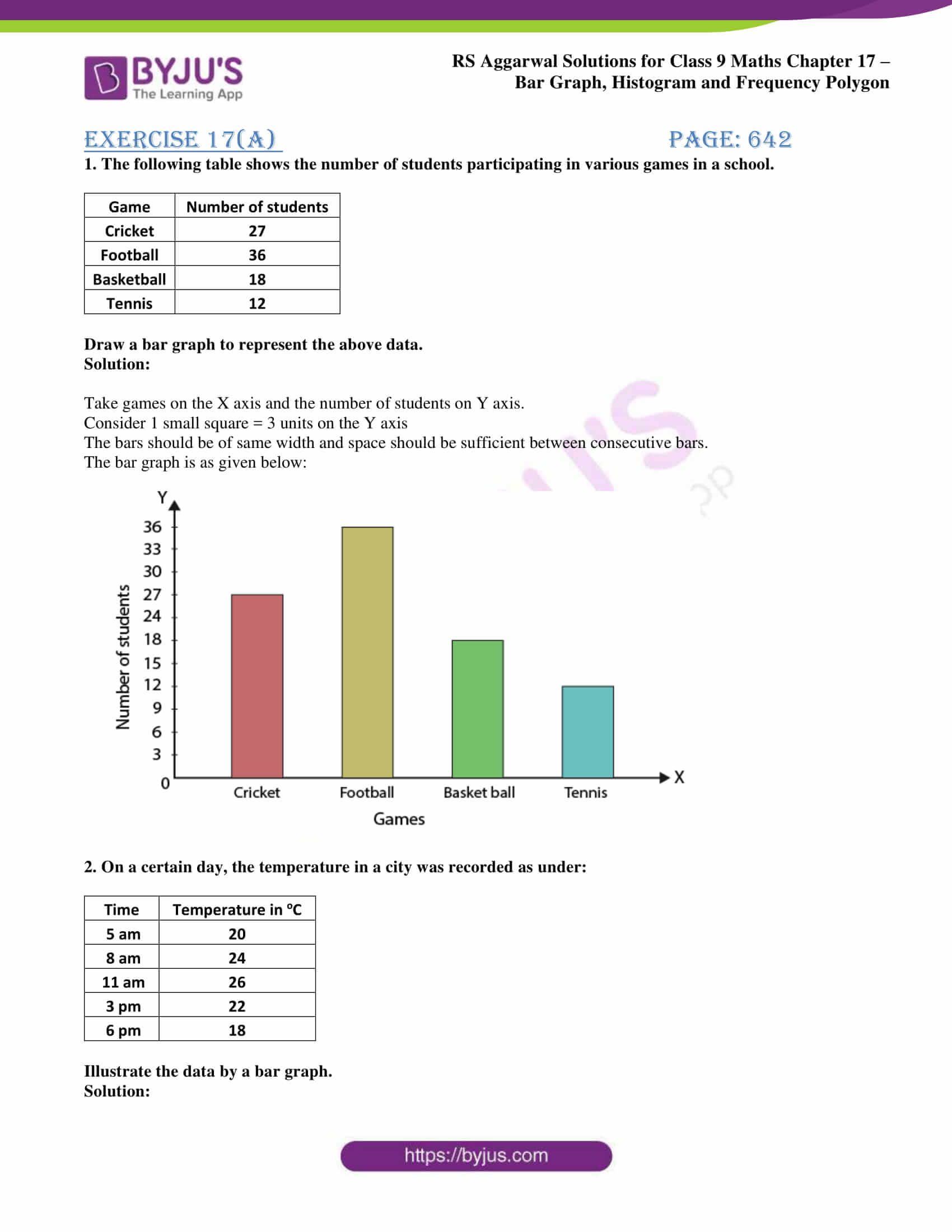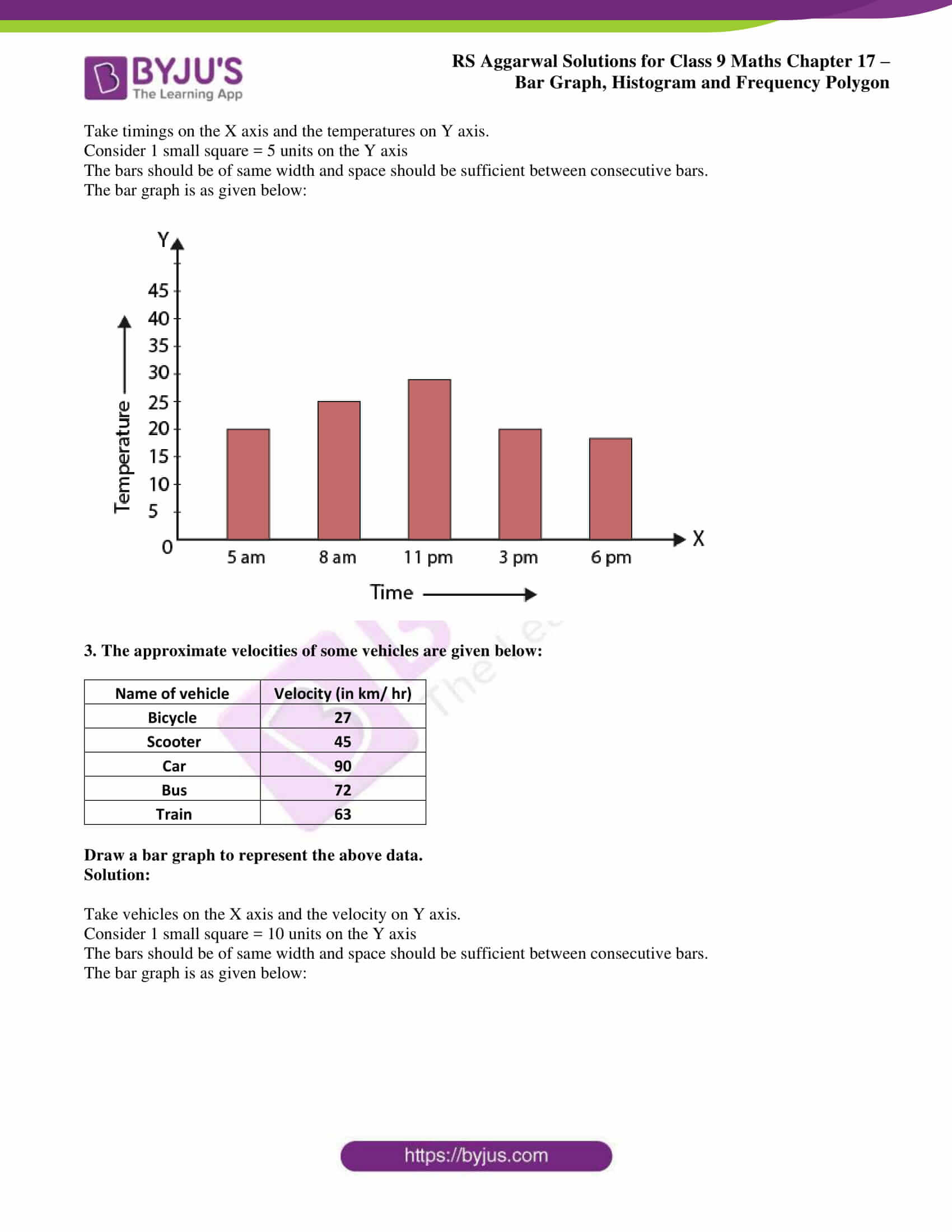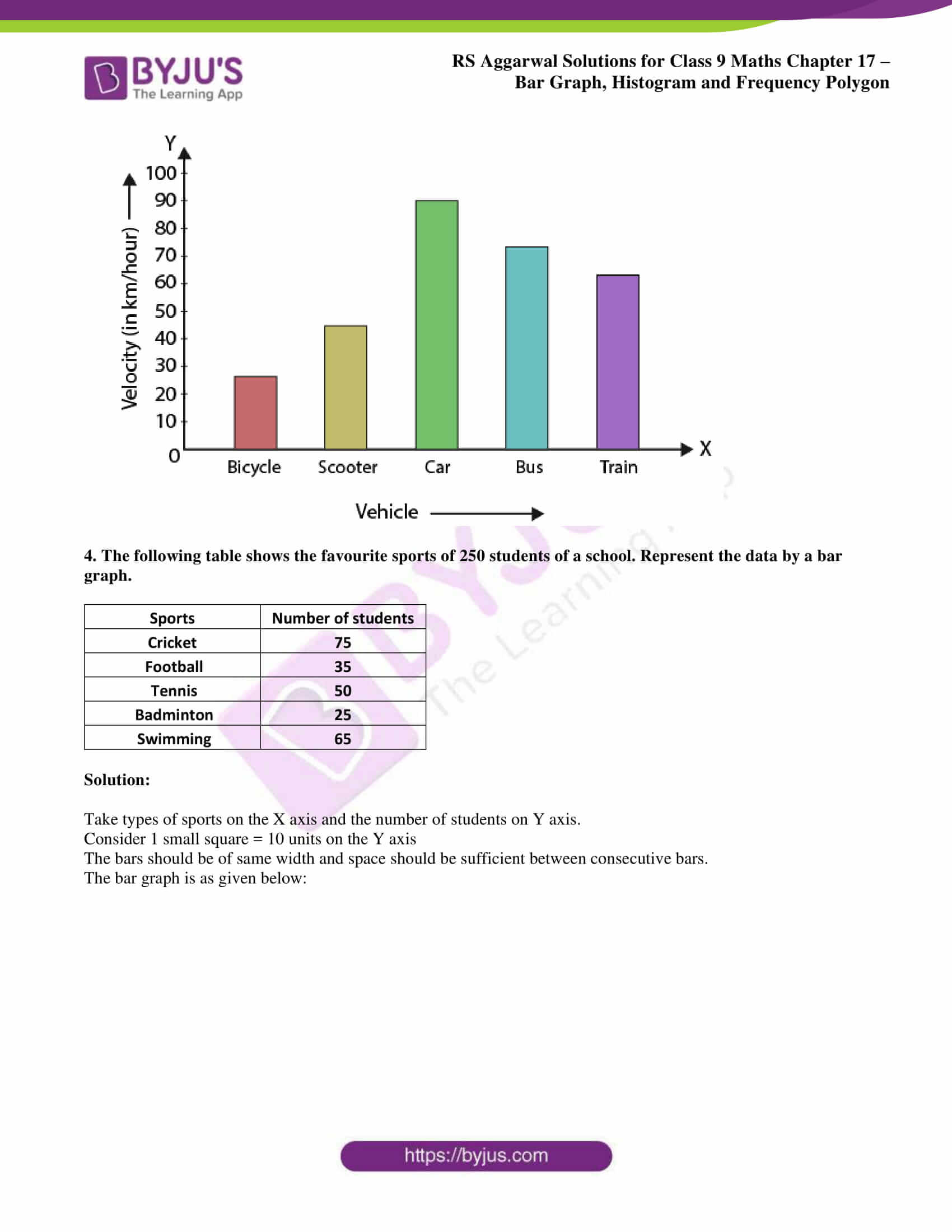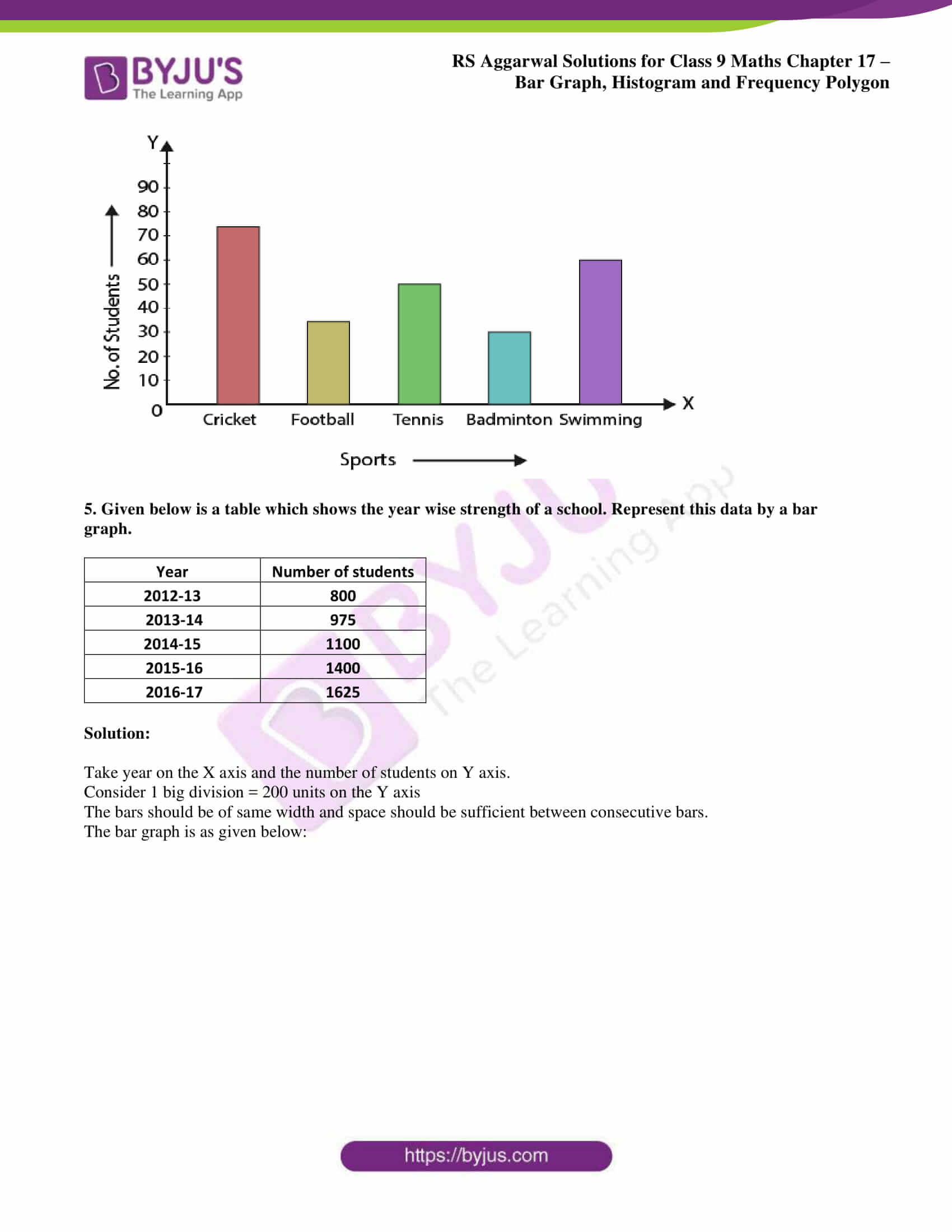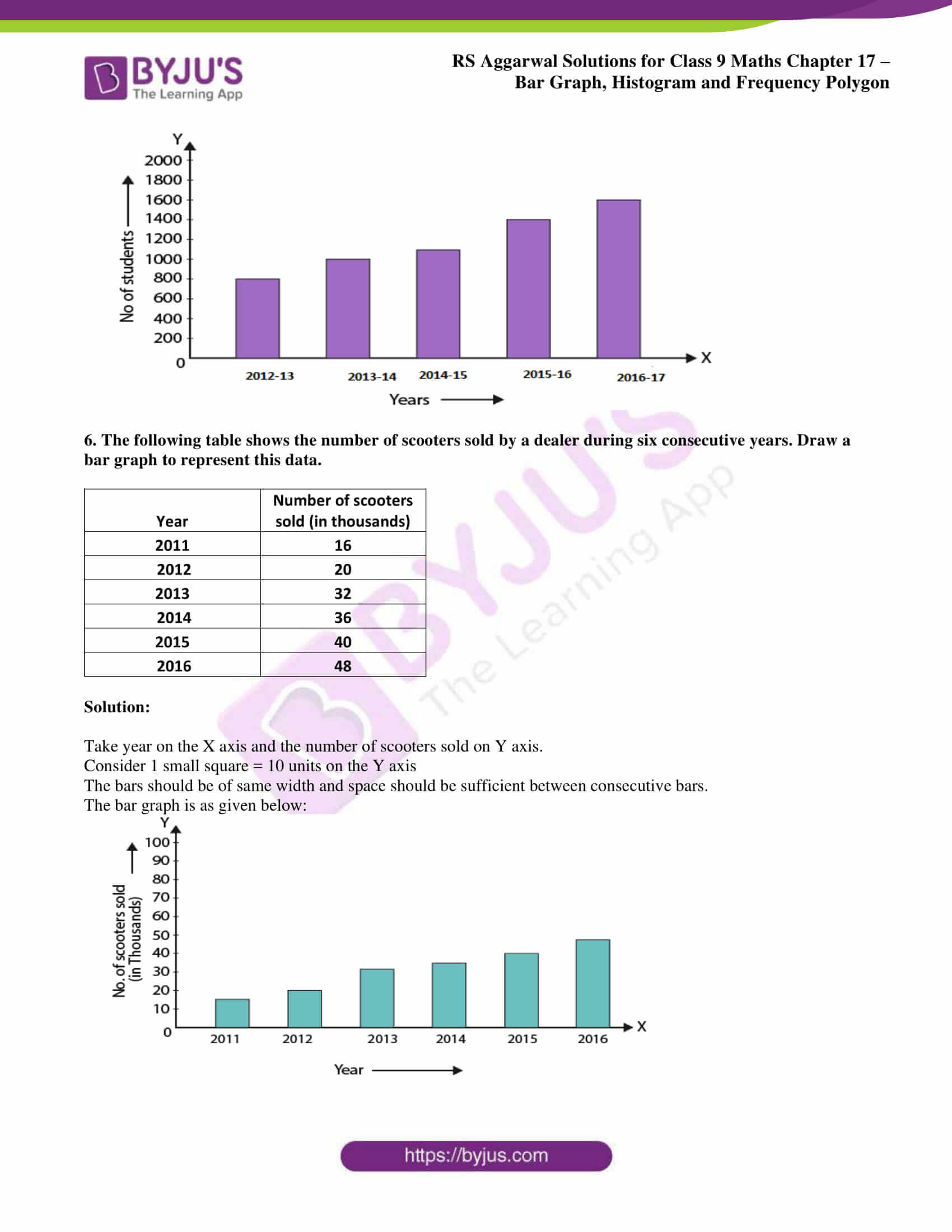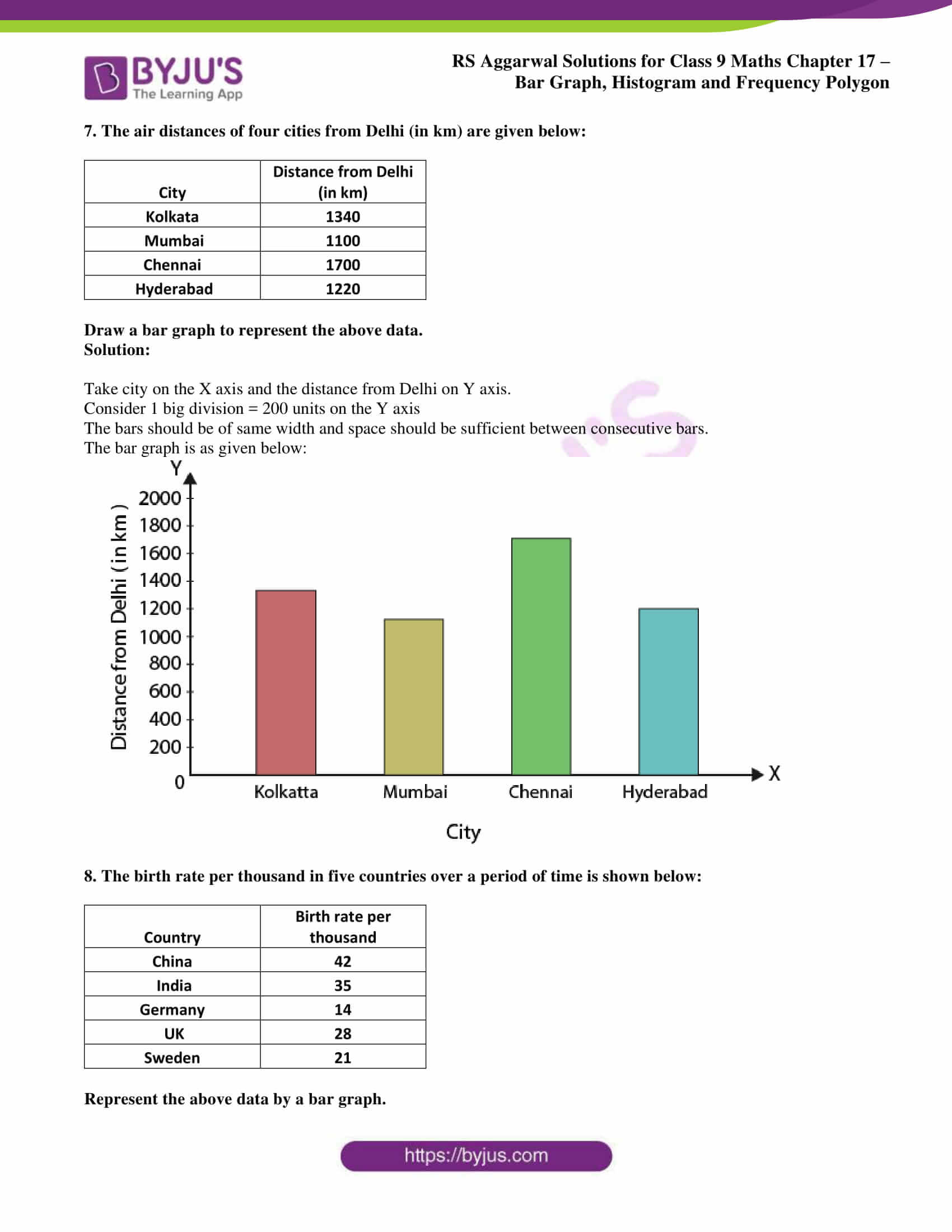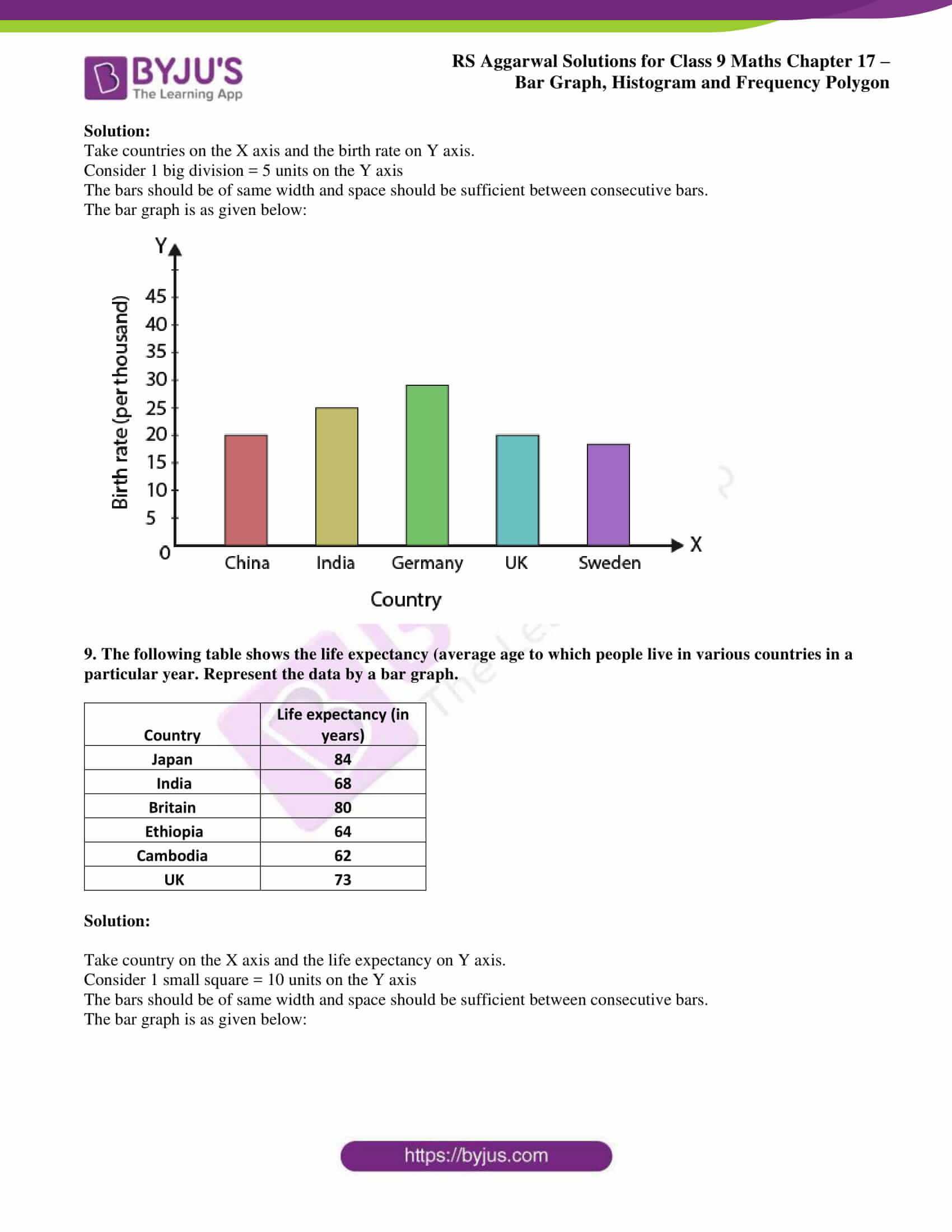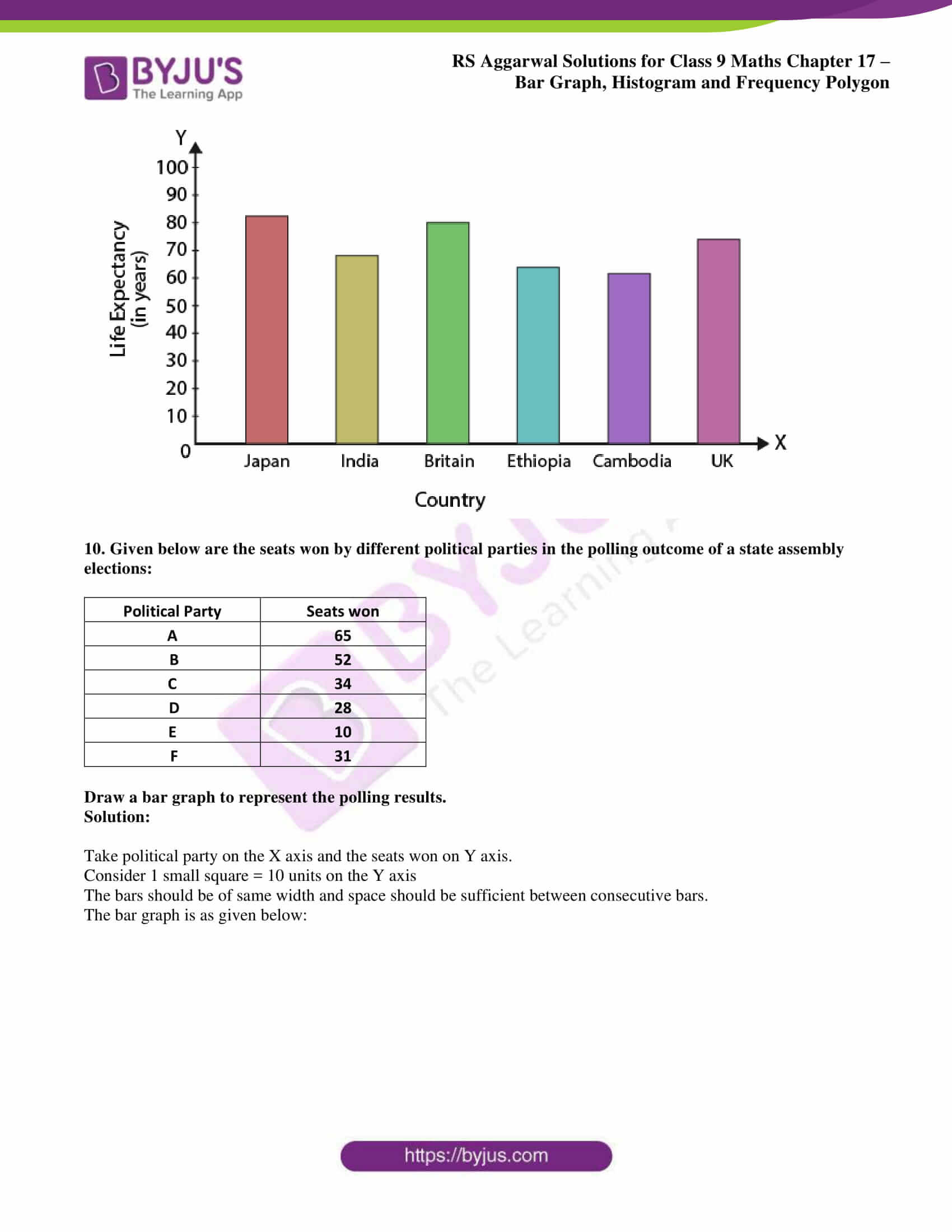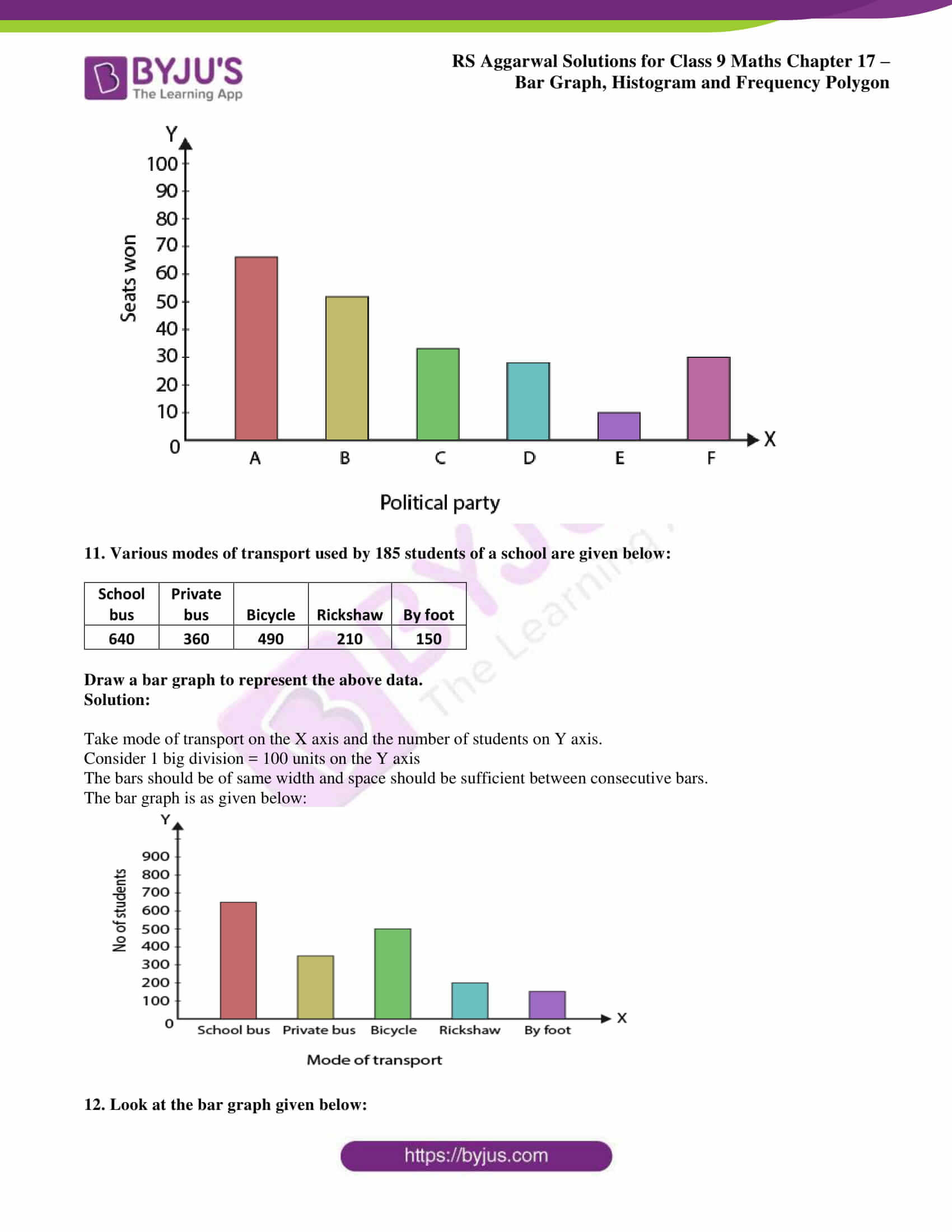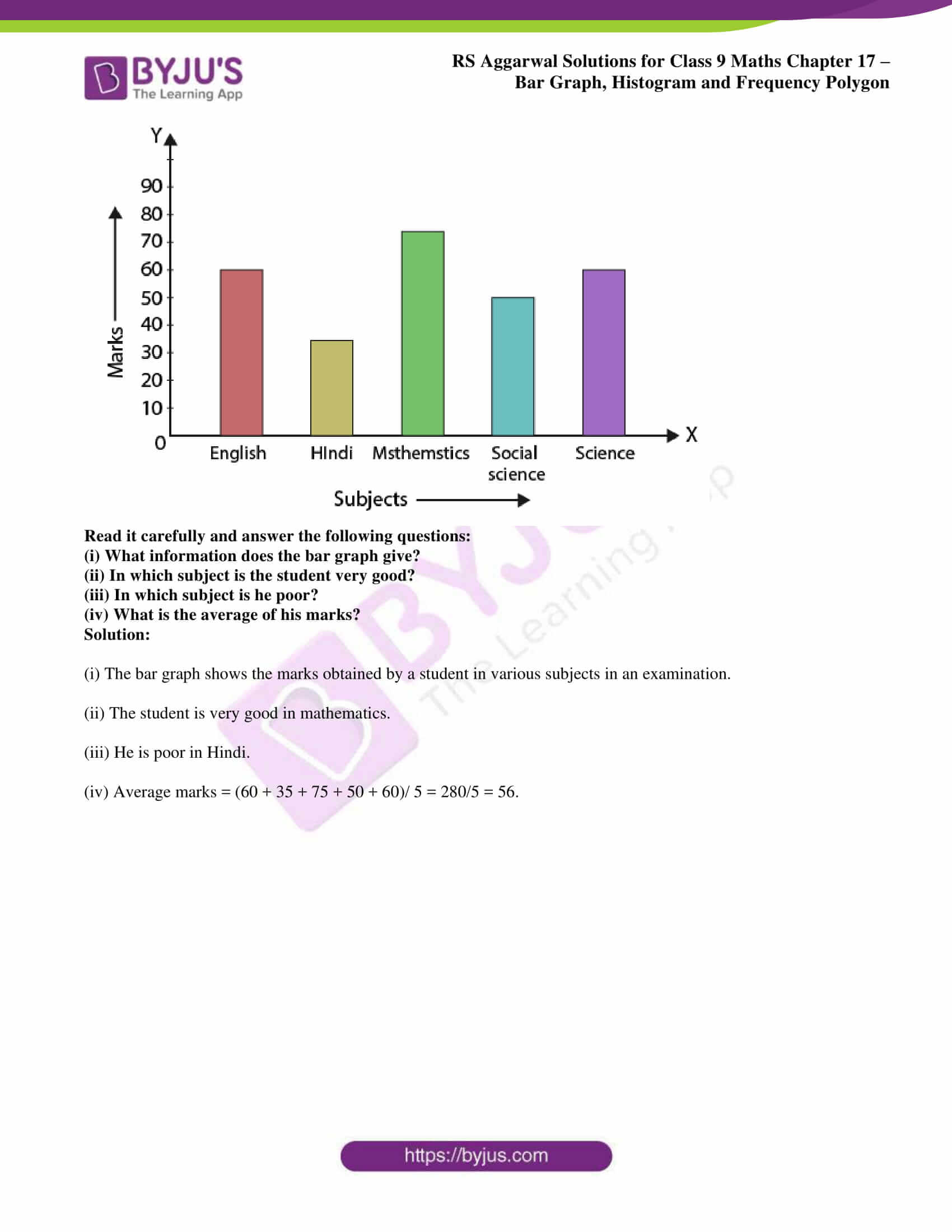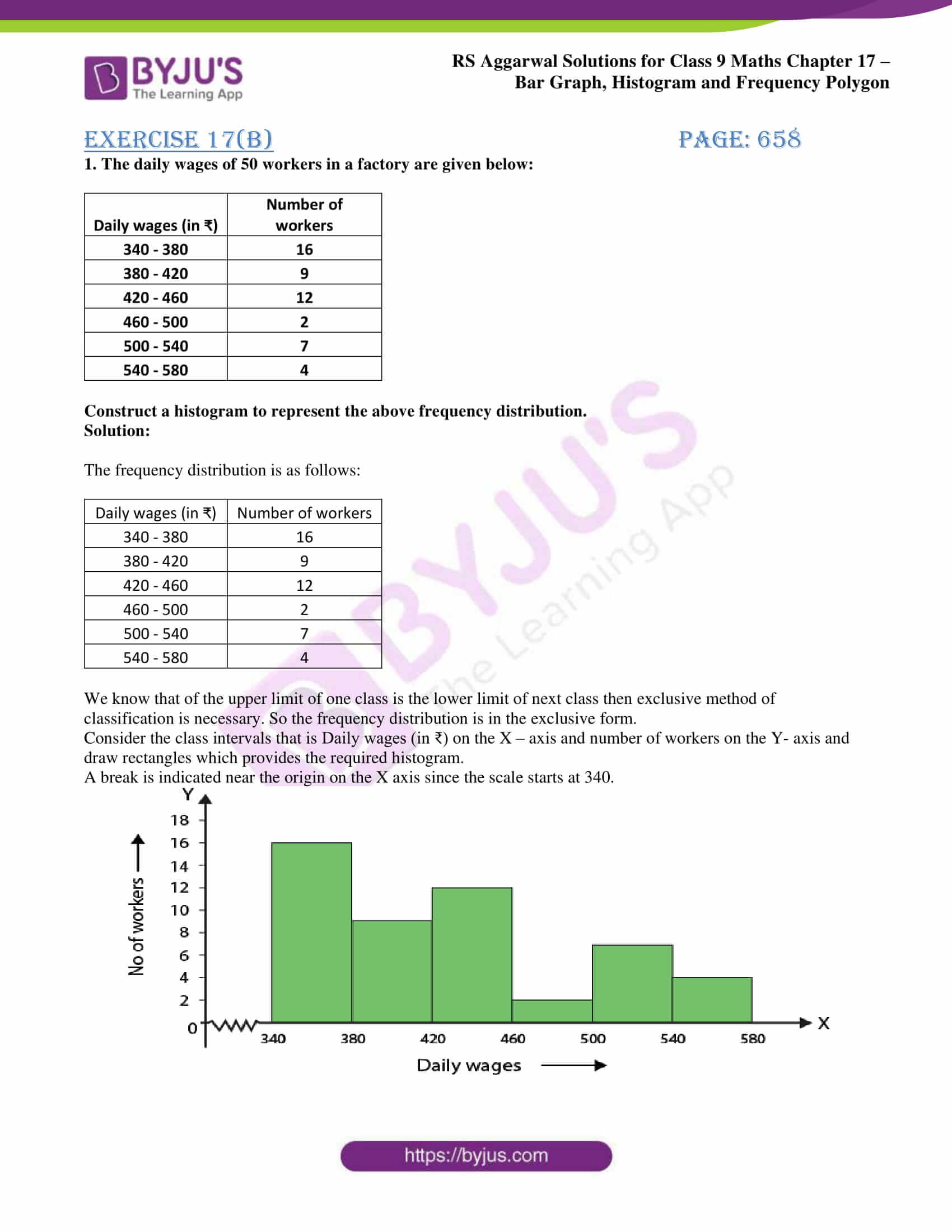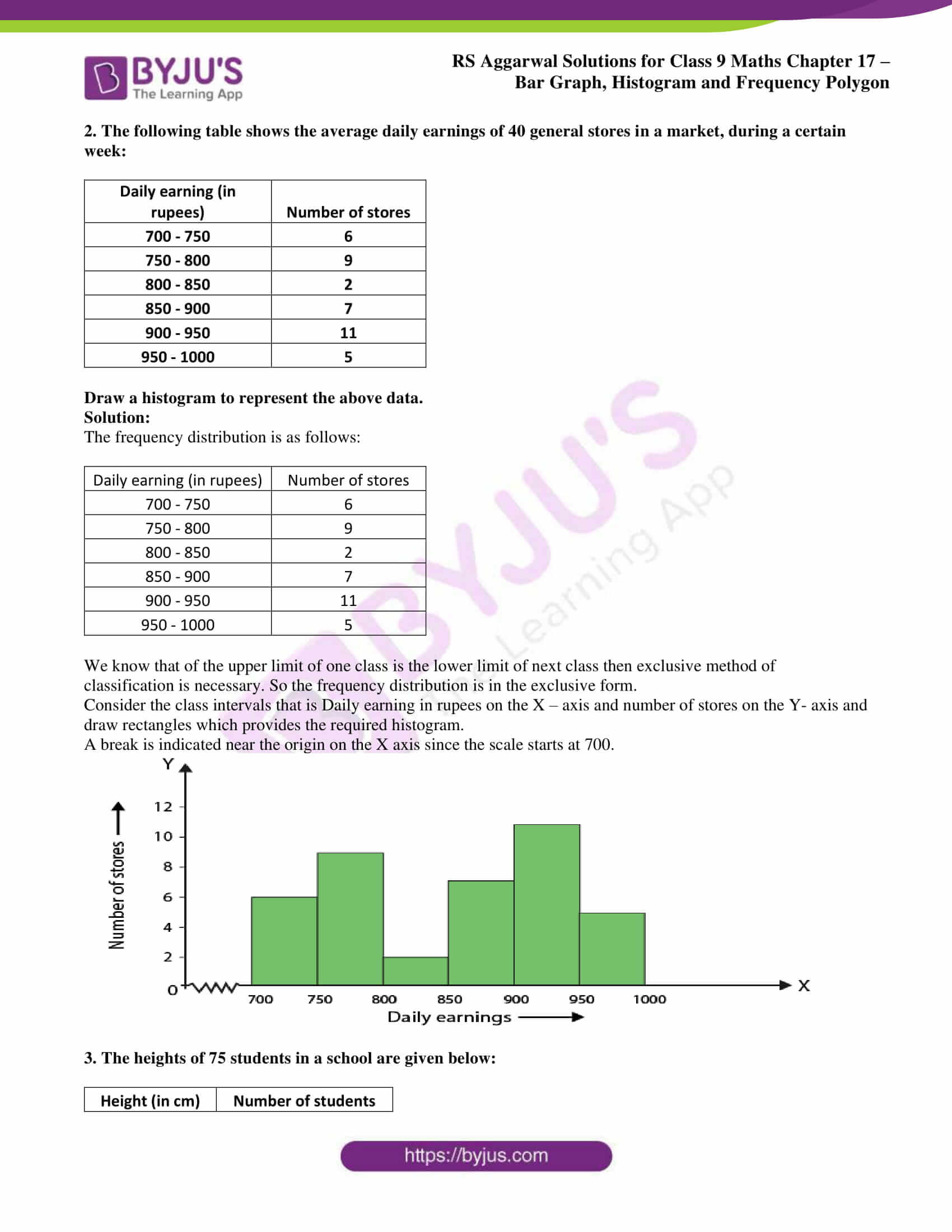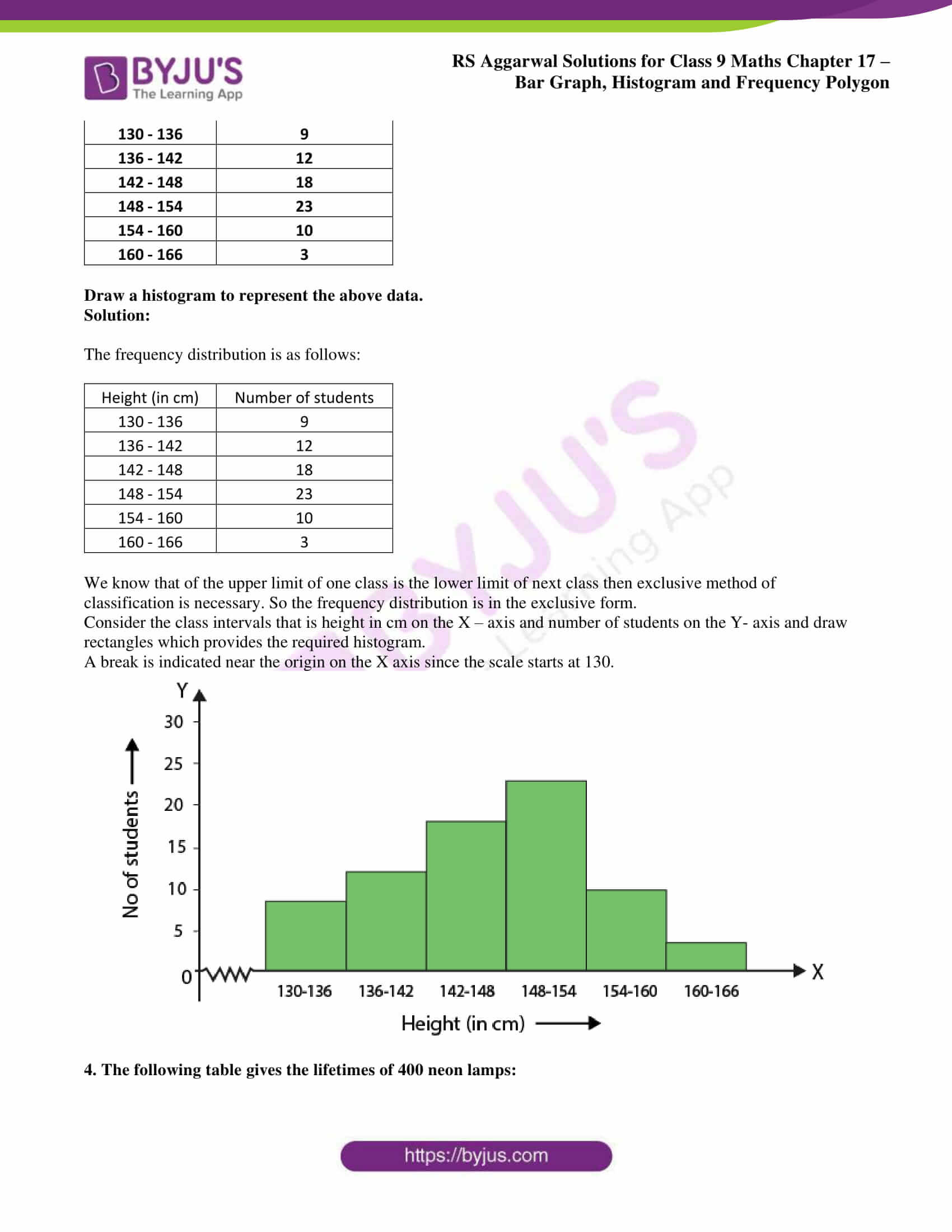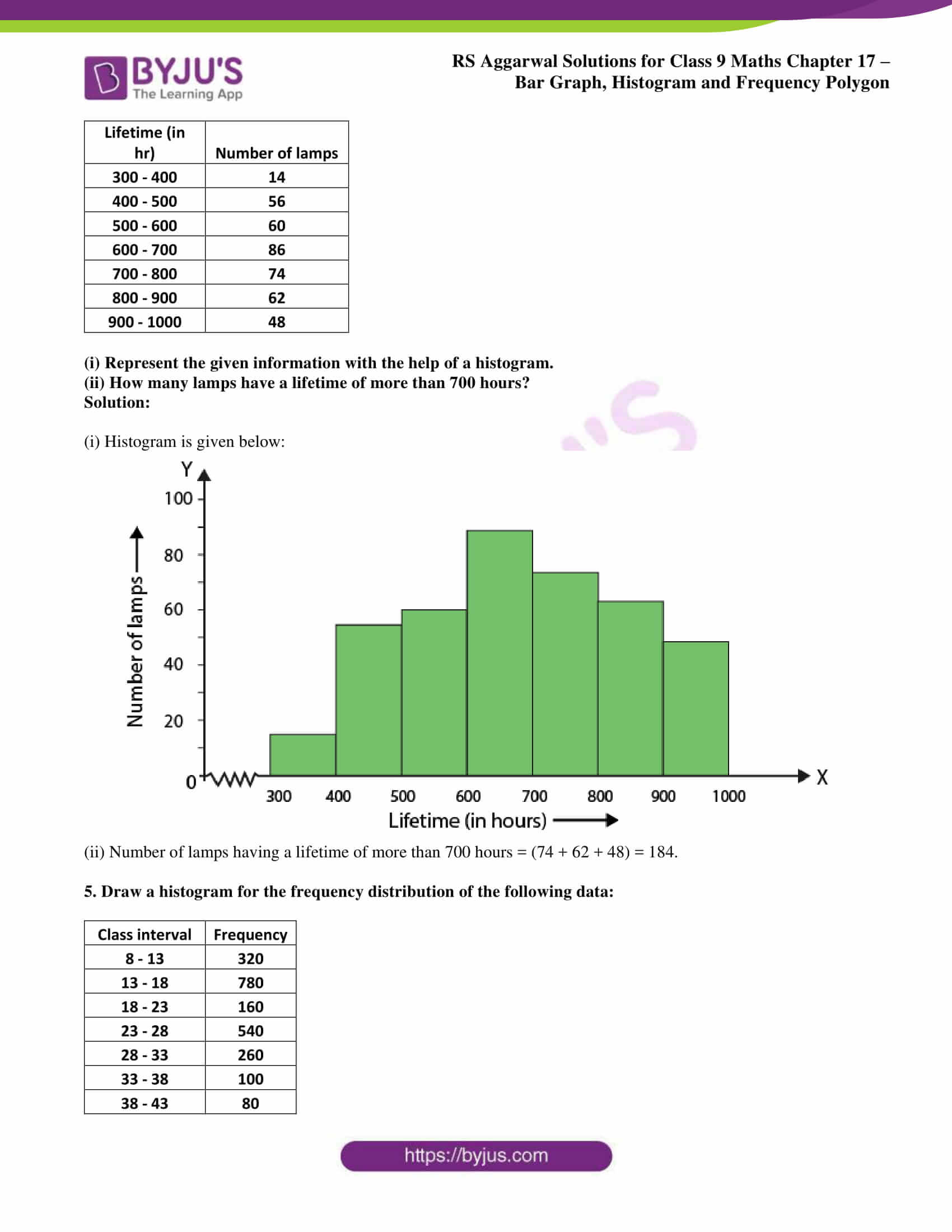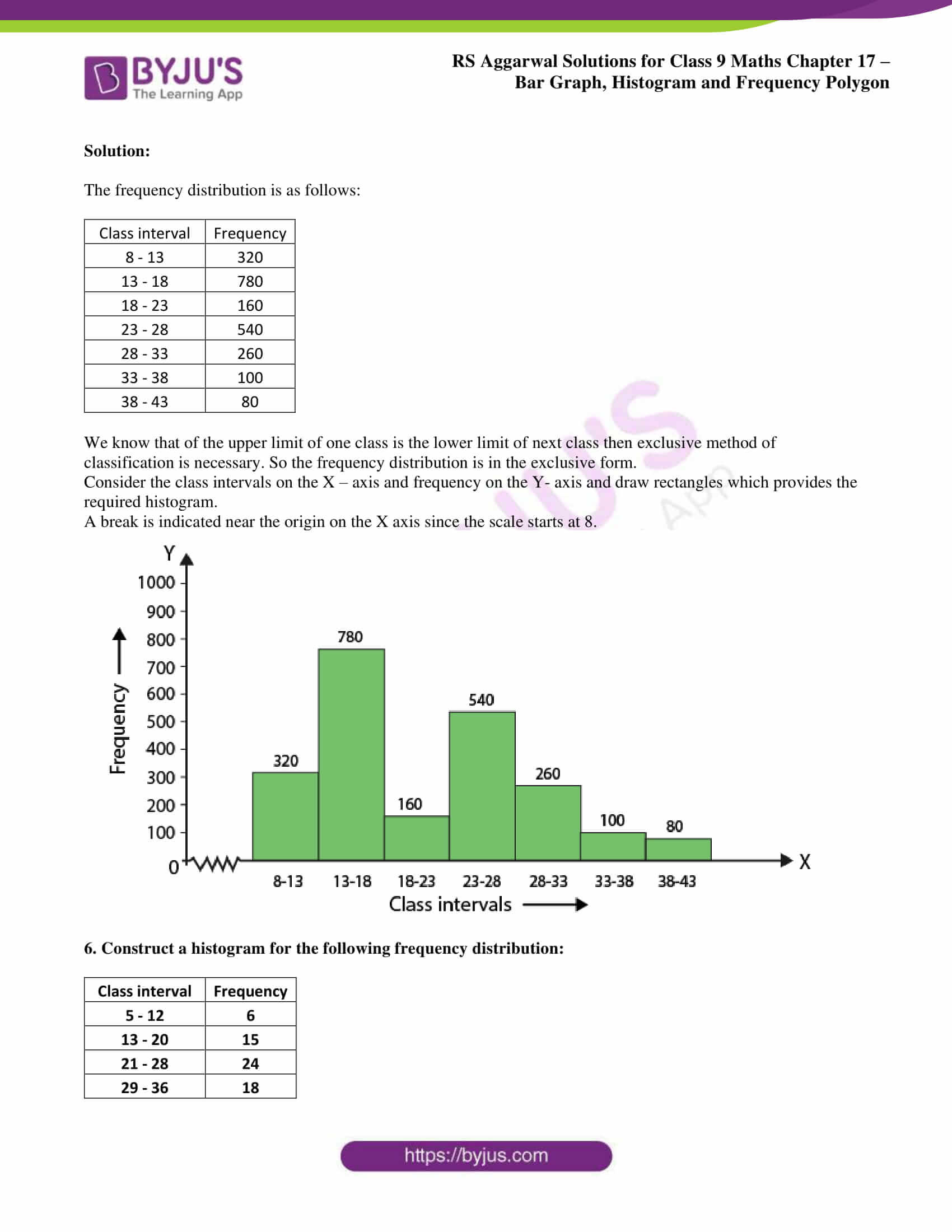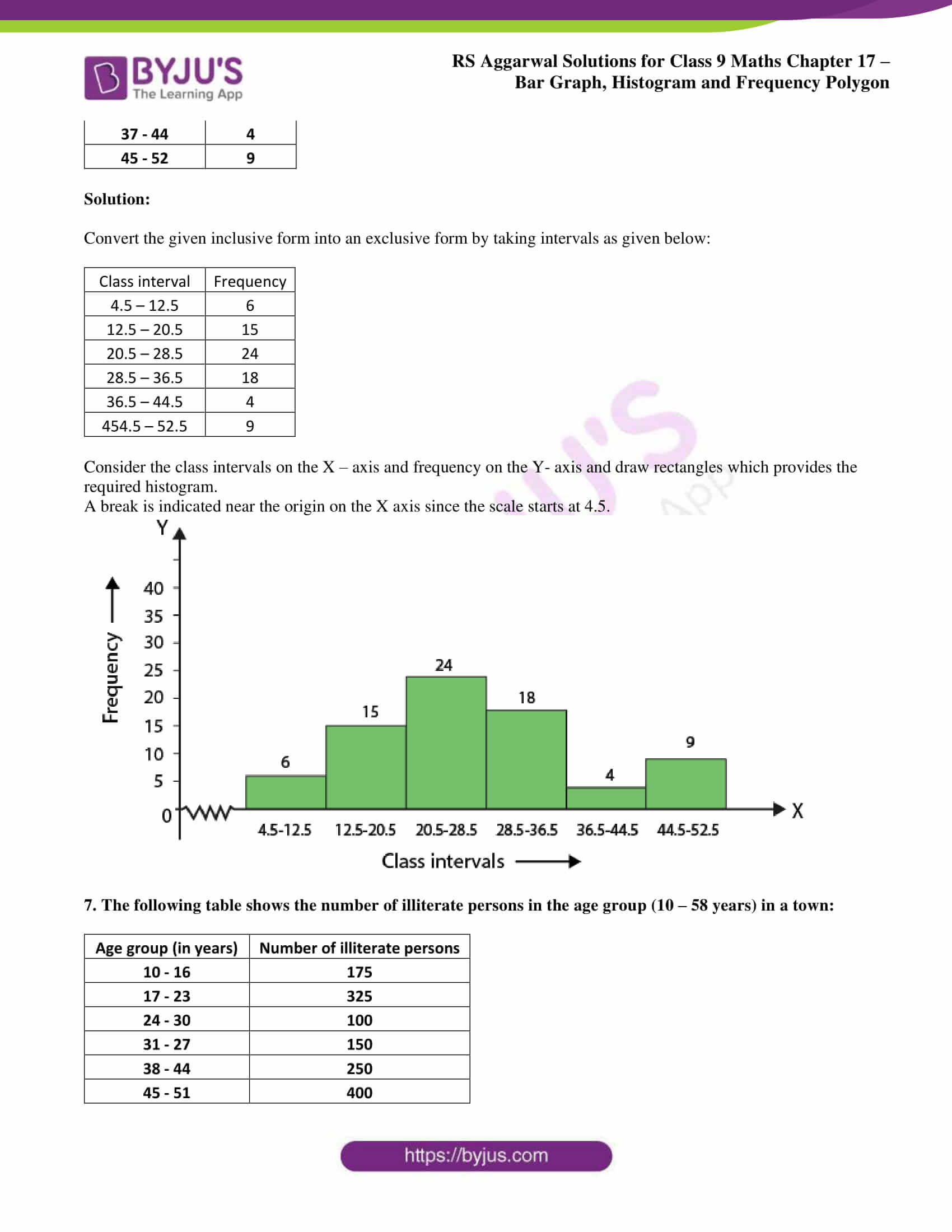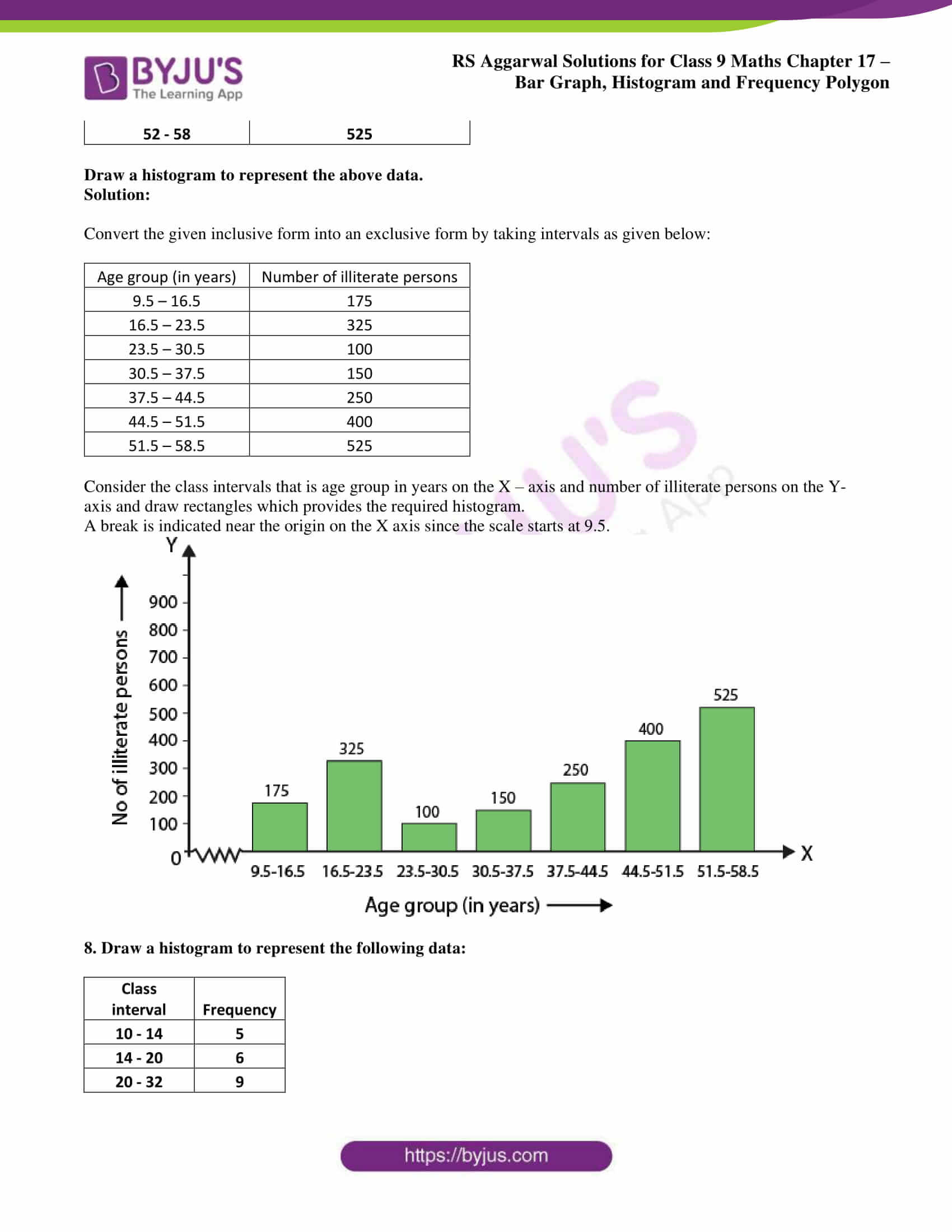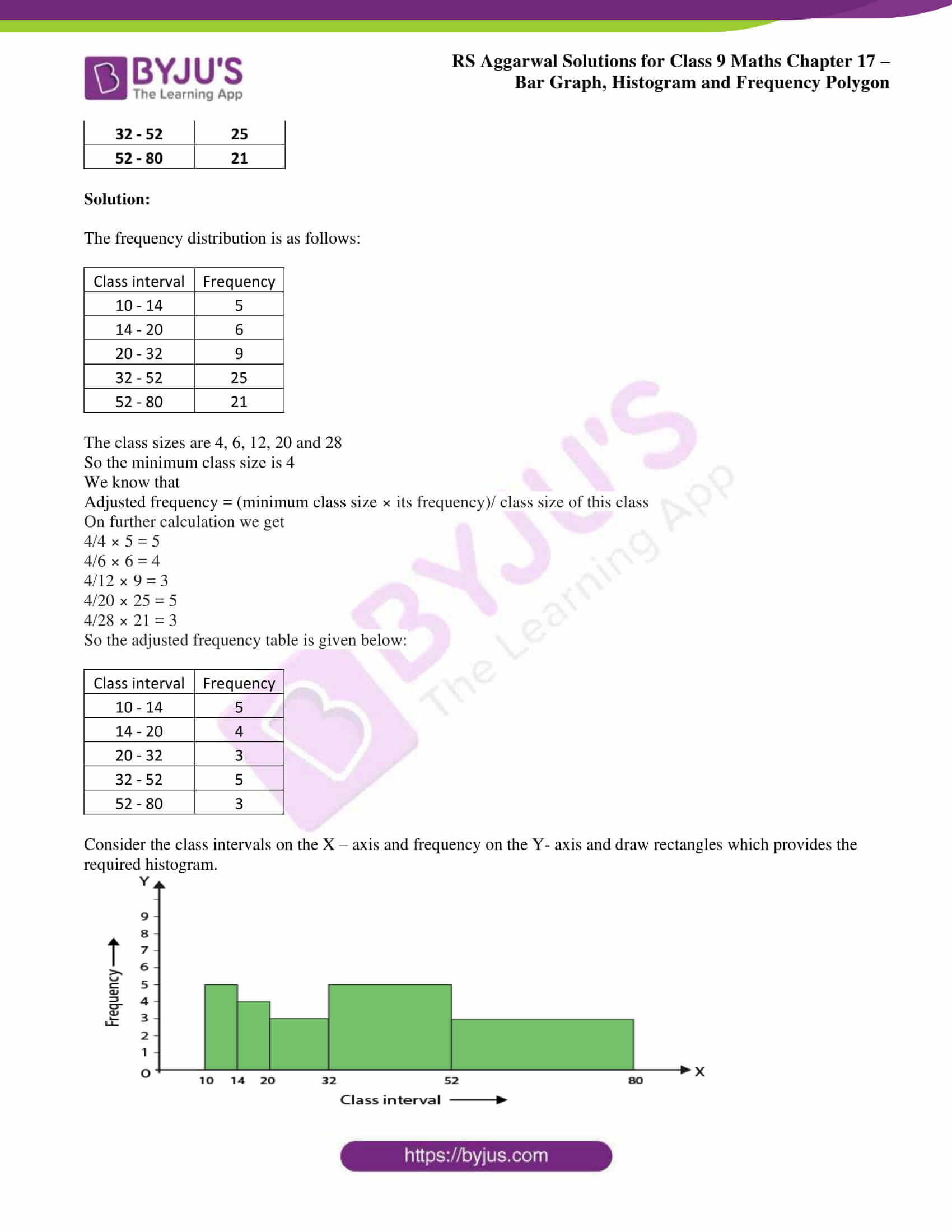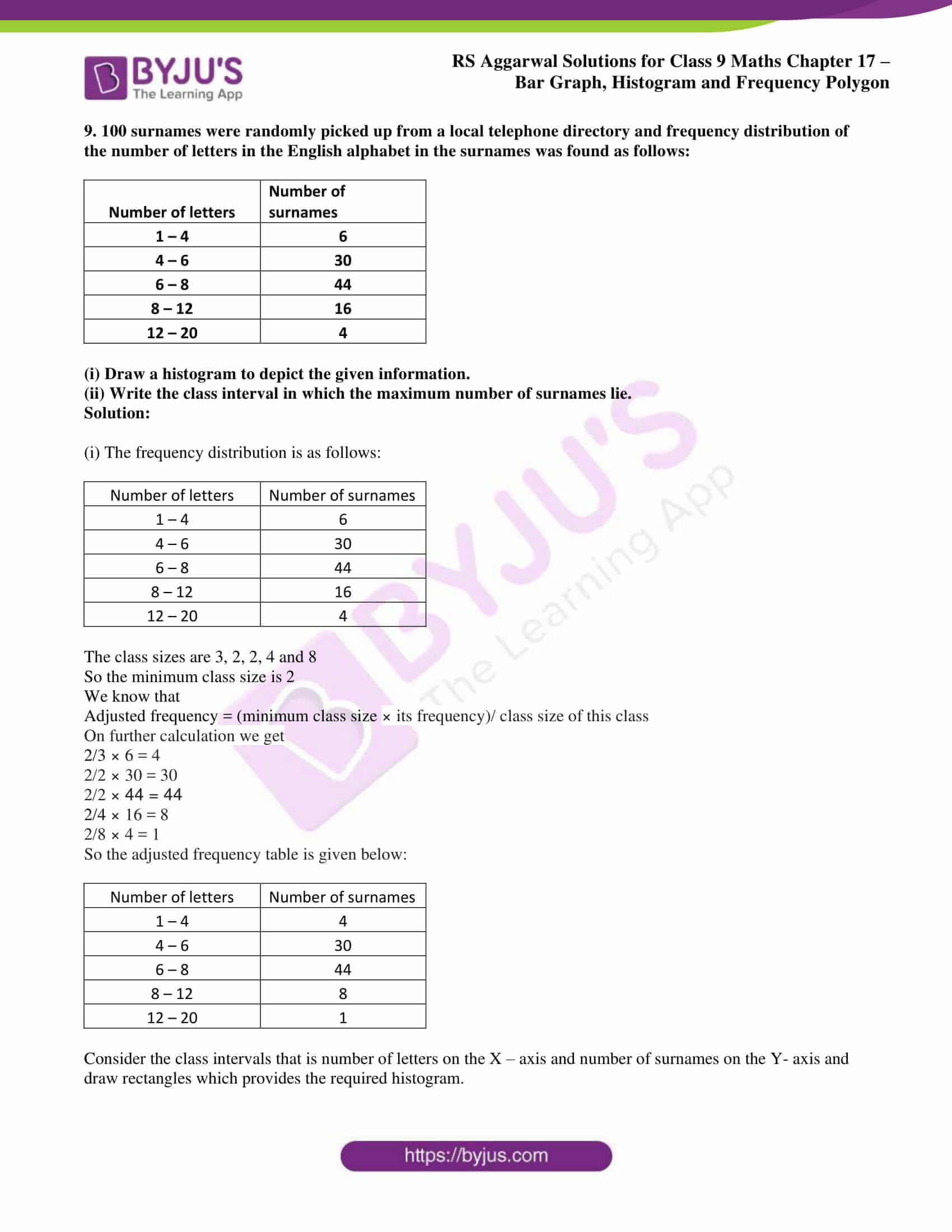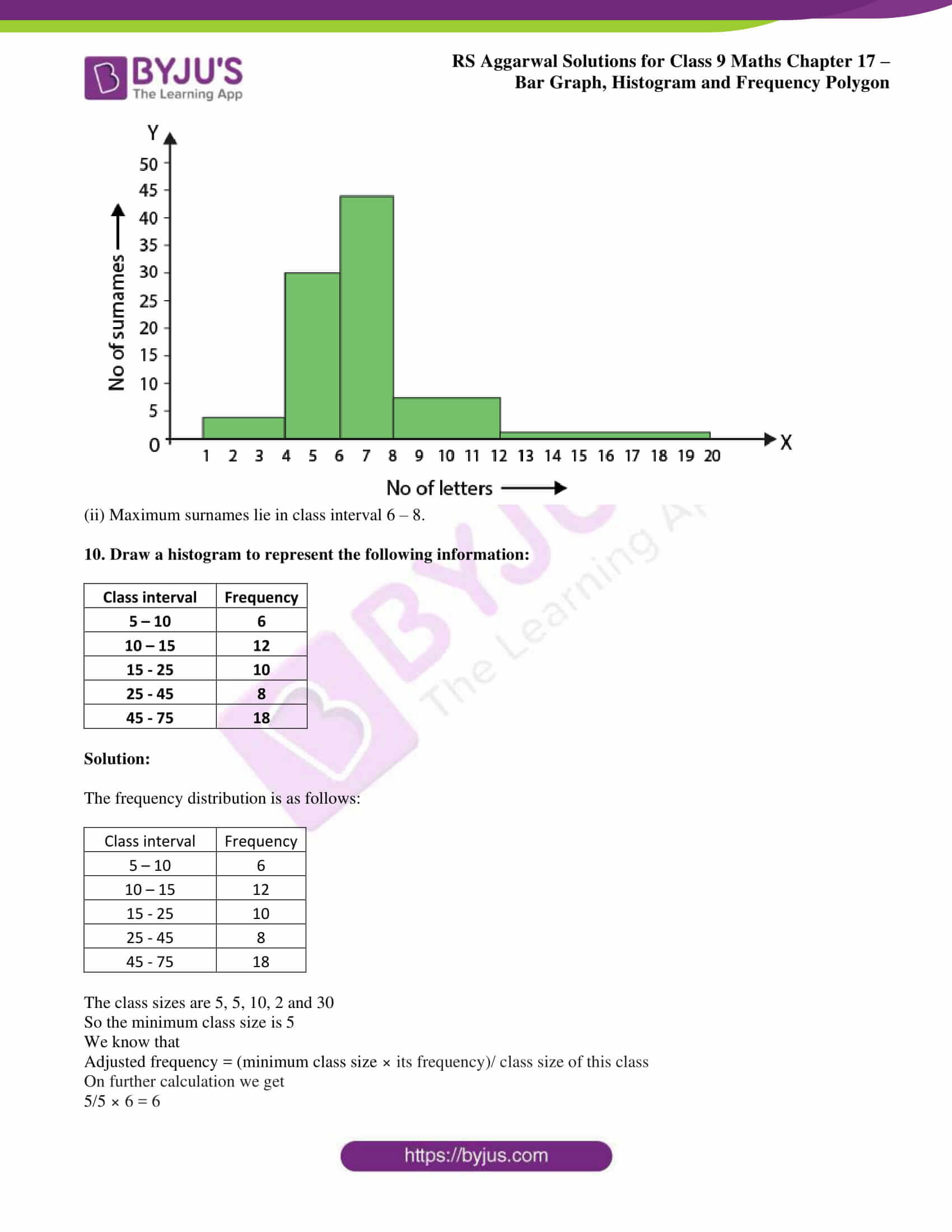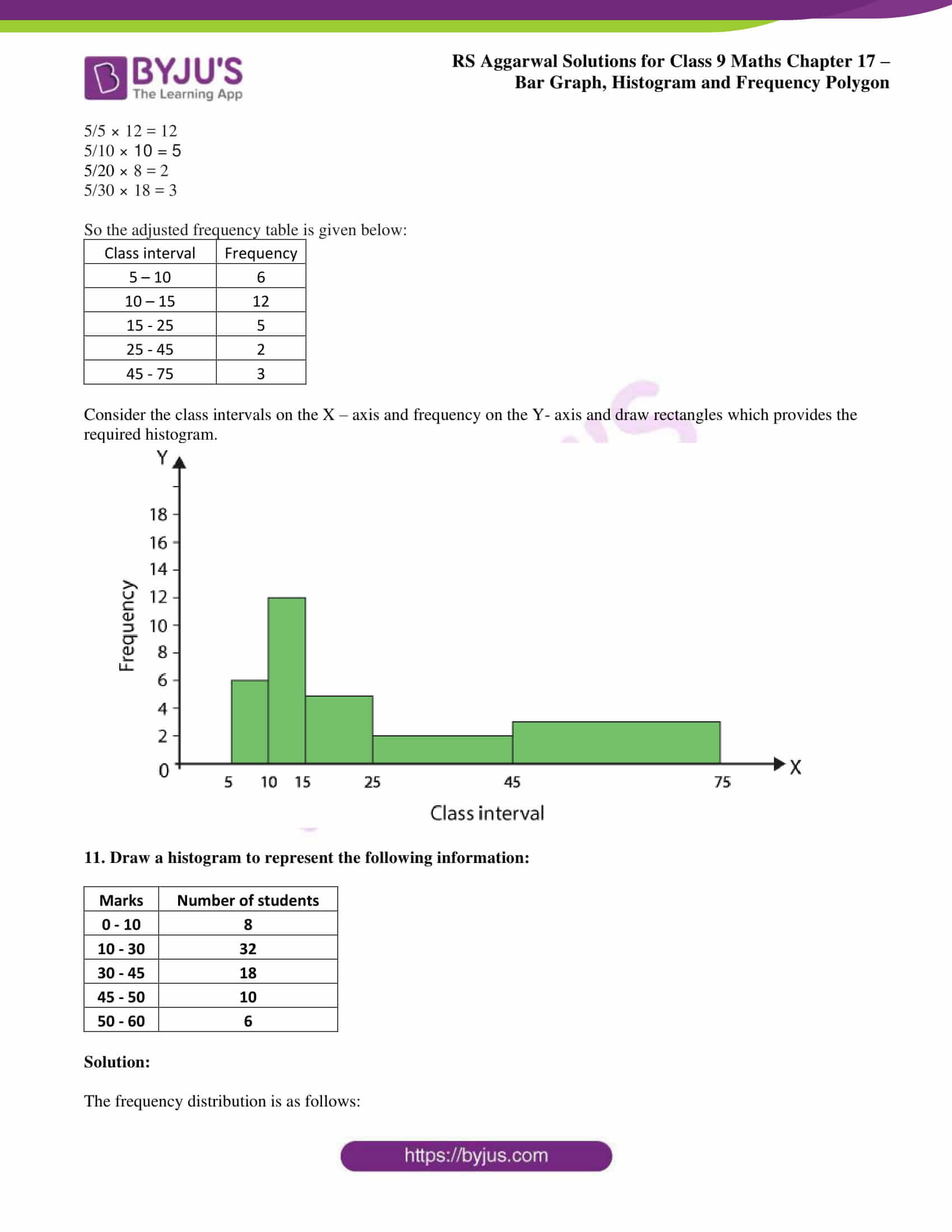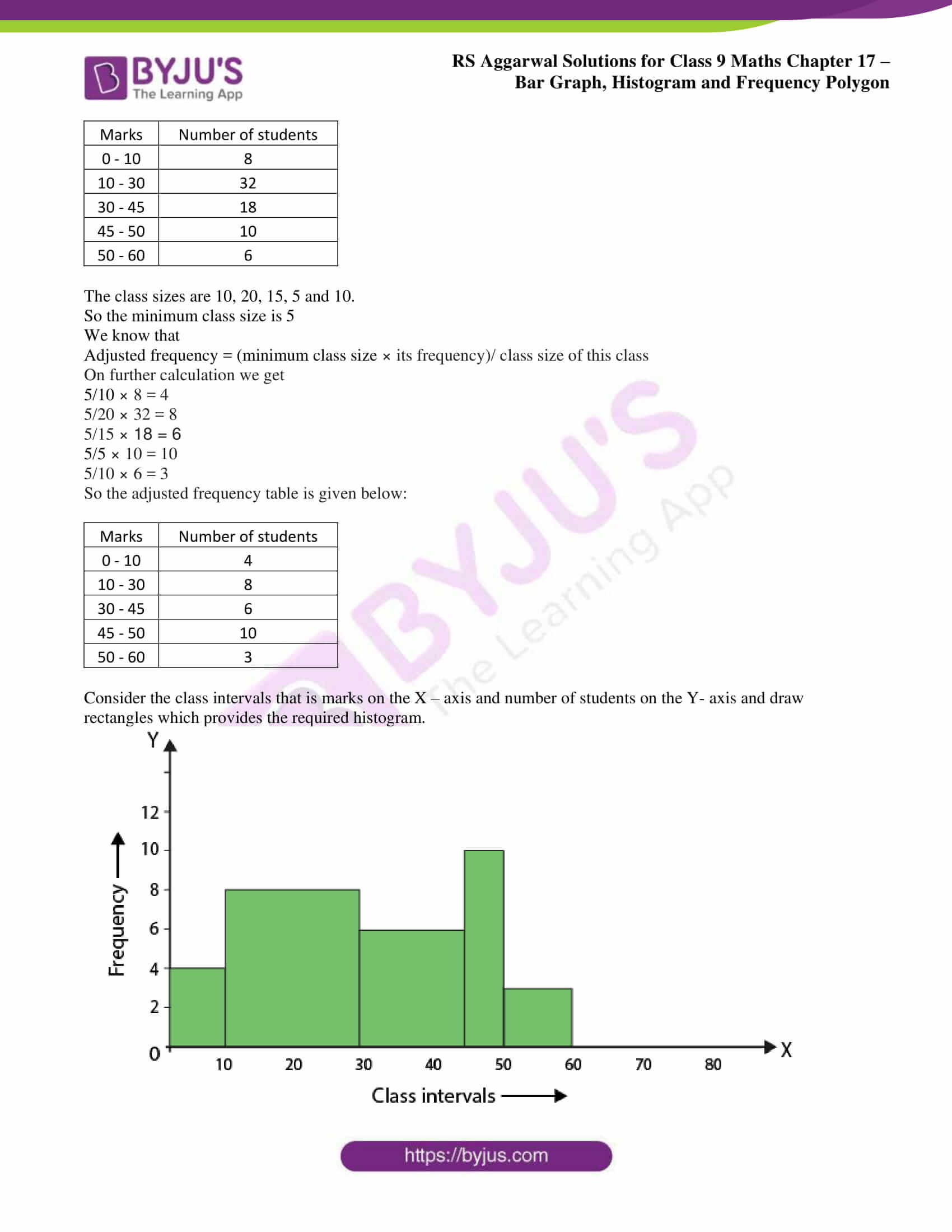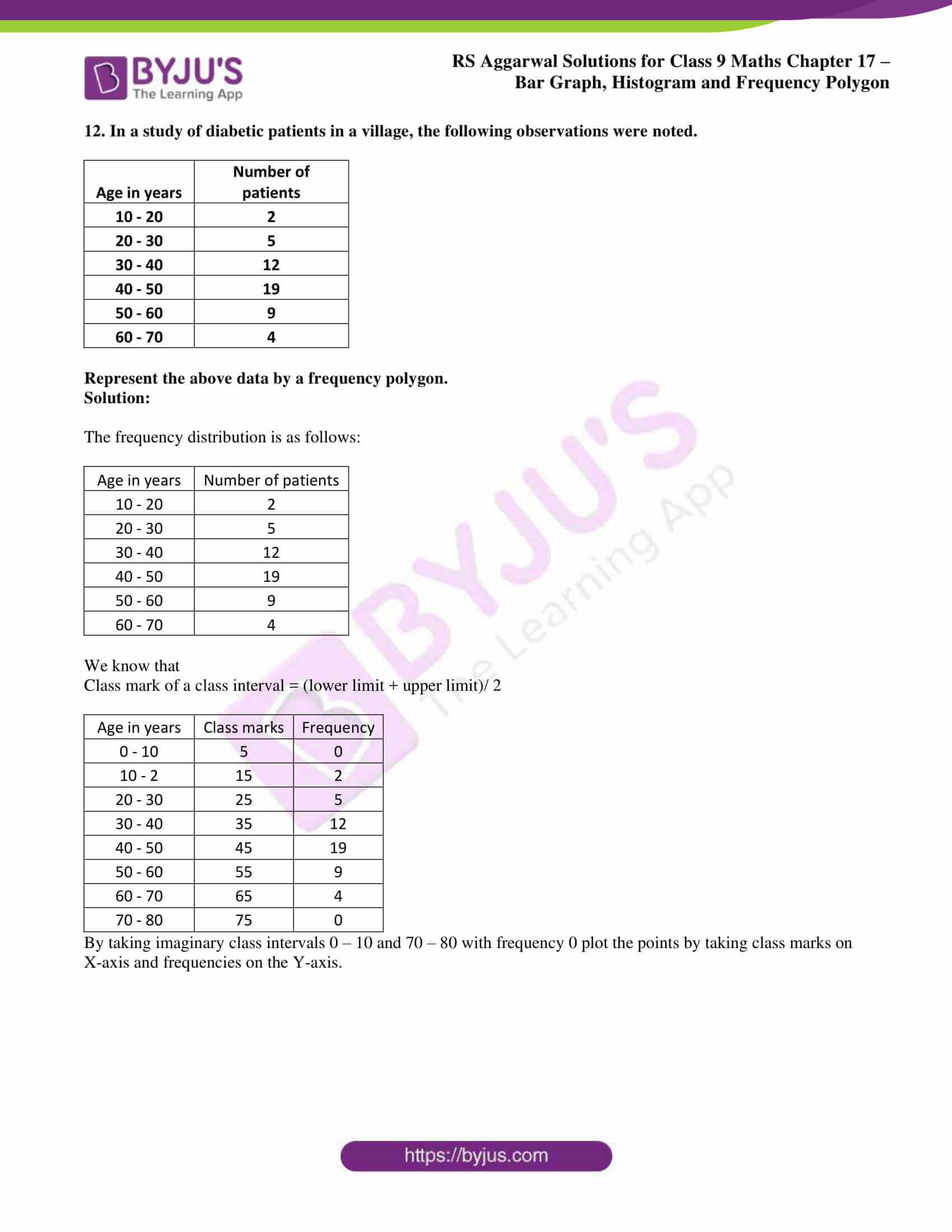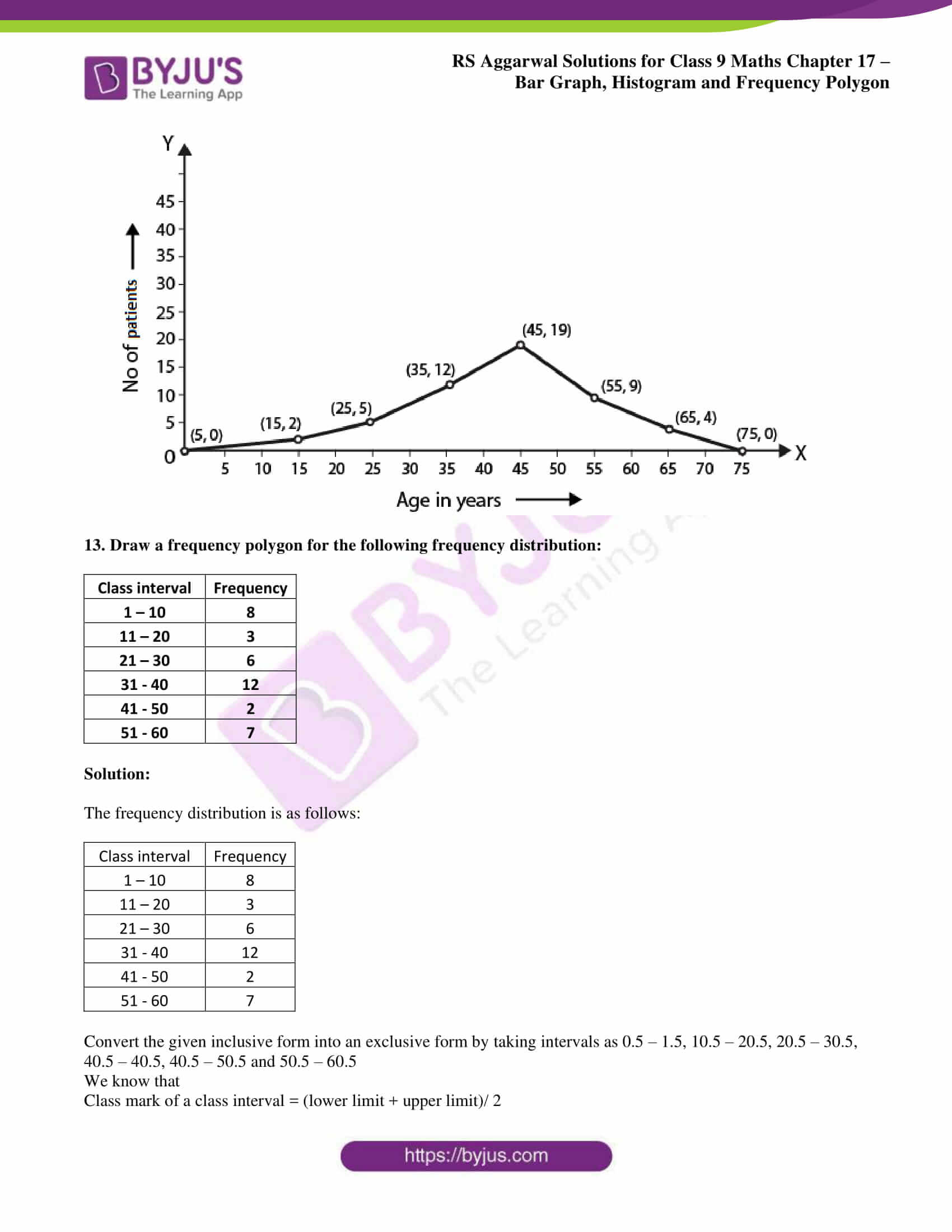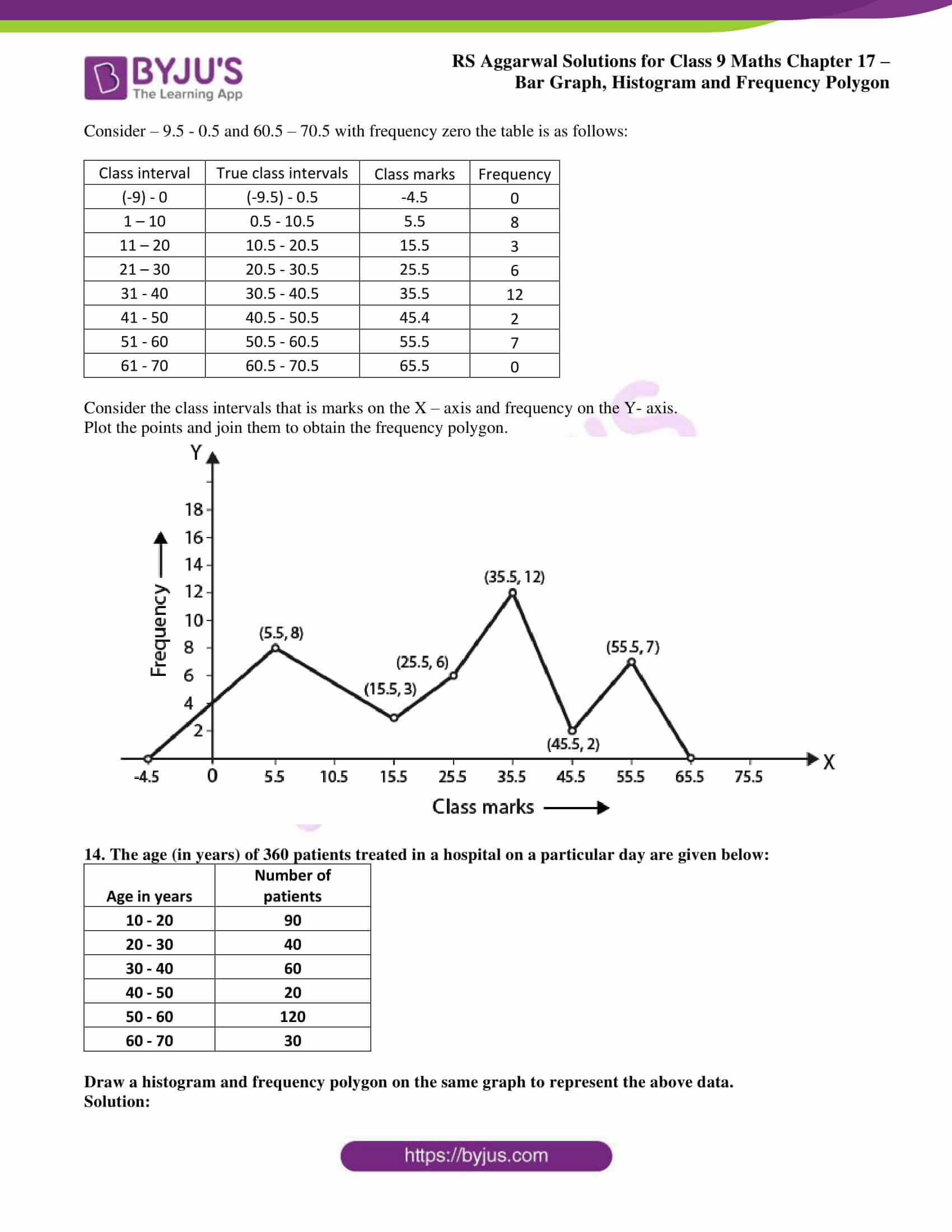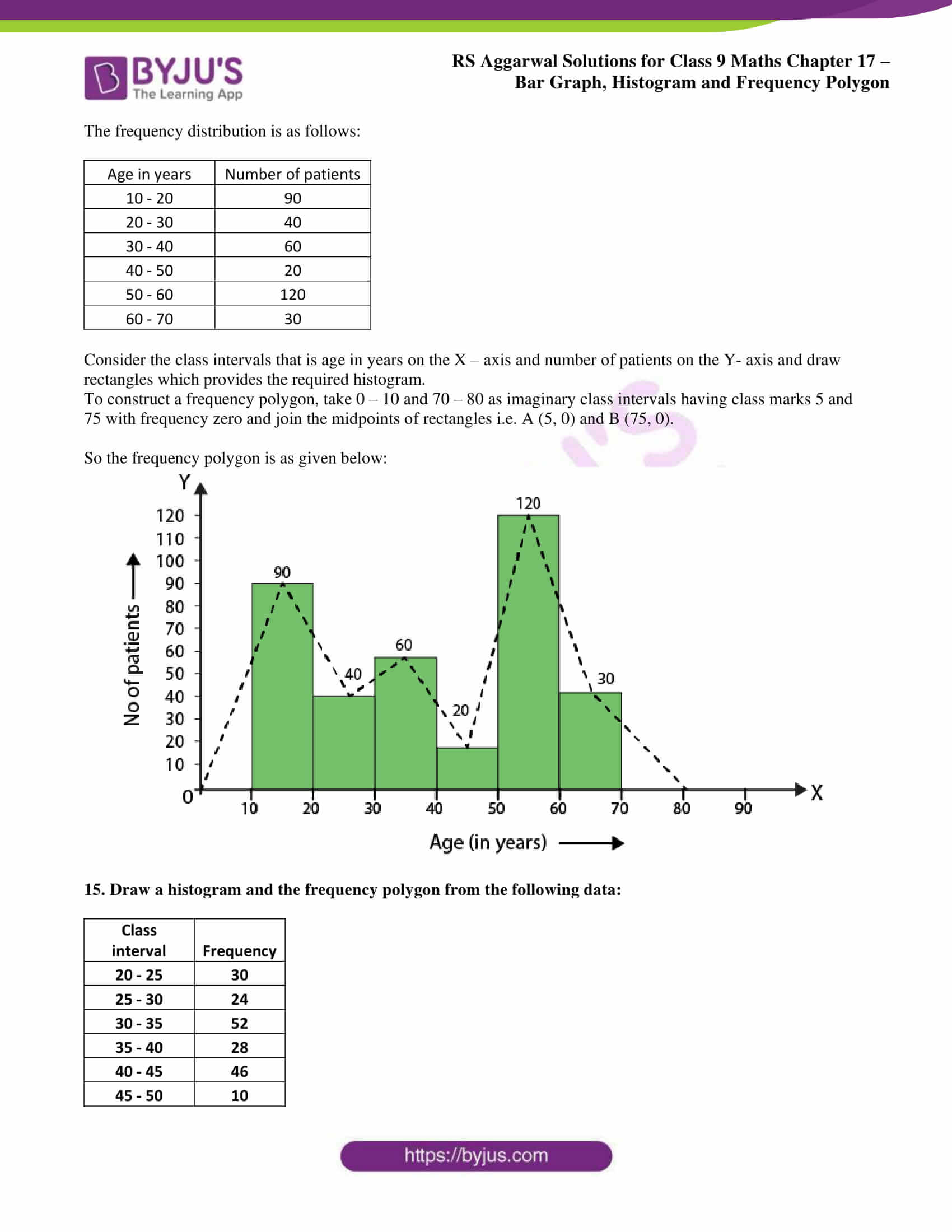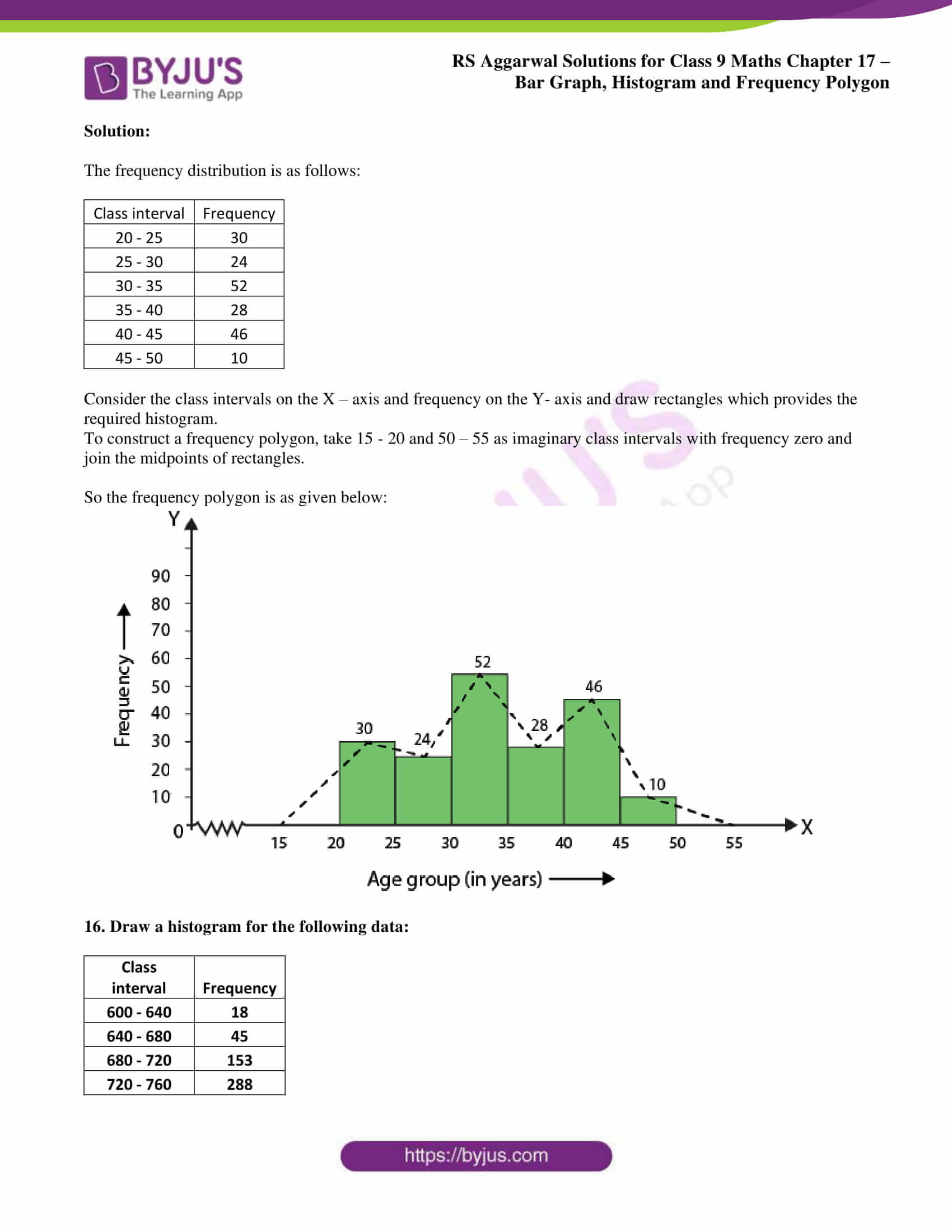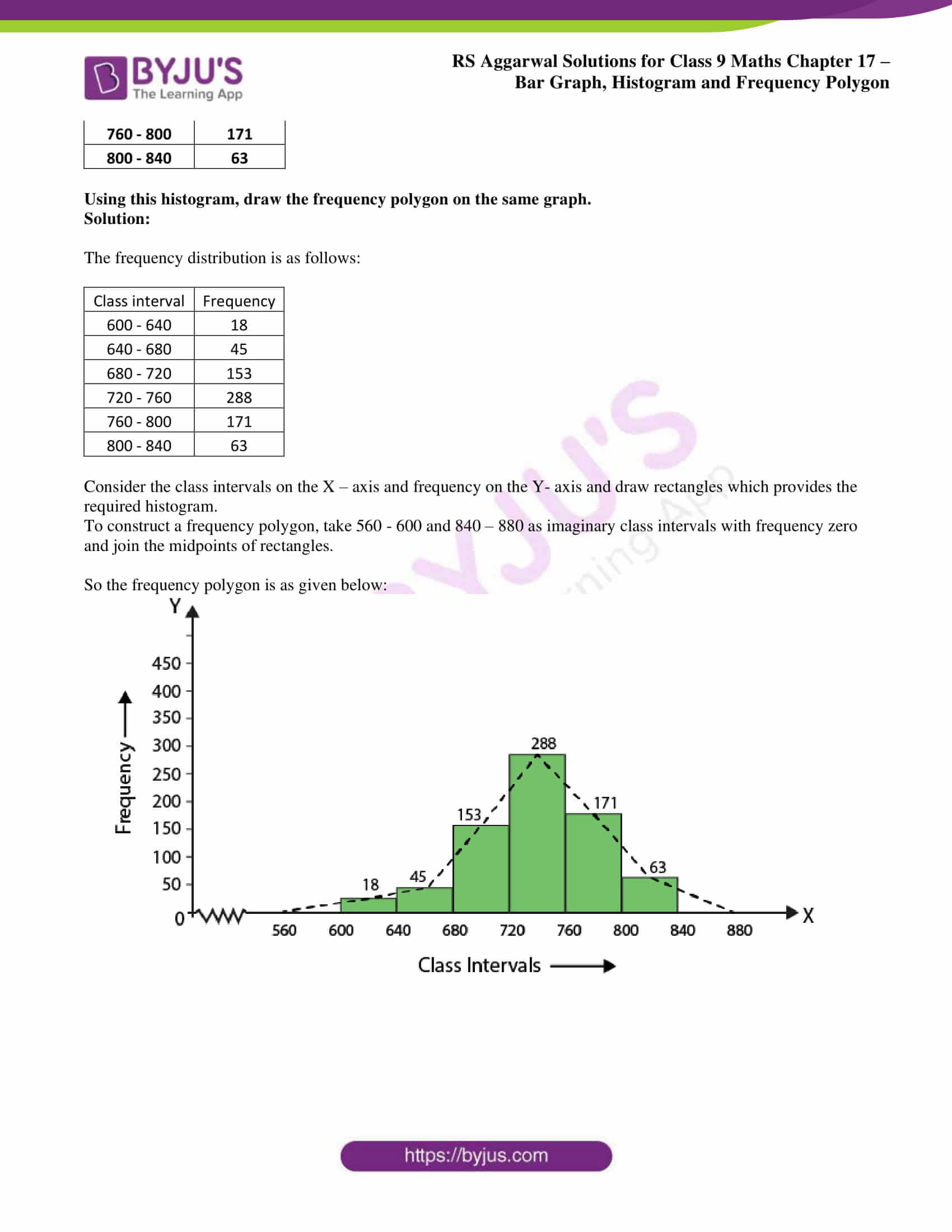### RS Aggarwal Solutions Class 9 Maths Chapter 17 – Exercise list

Exercise 17A Solutions 12 Questions

Exercise 17B Solutions 16 Questions

## Exercise 17(A) page: 642

1. The following table shows the number of students participating in various games in a school.

 Game Number of students Cricket 27 Football 36 Basketball 18 Tennis 12

Draw a bar graph to represent the above data.

Solution:

Take games on the X axis and the number of students on Y axis.

Consider 1 small square = 3 units on the Y axis

The bars should be of same width and space should be sufficient between consecutive bars.

The bar graph is as given below: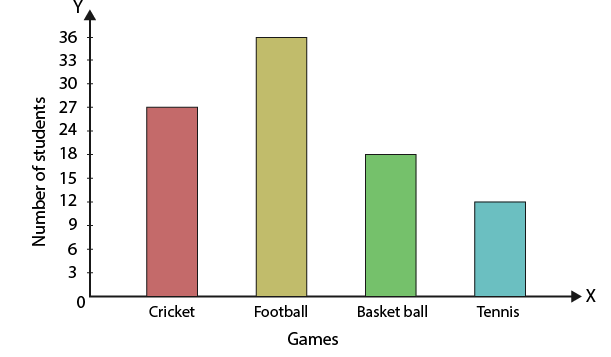2. On a certain day, the temperature in a city was recorded as under:

 Time Temperature in oC 5 am 20 8 am 24 11 am 26 3 pm 22 6 pm 18

Illustrate the data by a bar graph.

Solution:

Take timings on the X axis and the temperatures on Y axis.

Consider 1 small square = 5 units on the Y axis

The bars should be of same width and space should be sufficient between consecutive bars.

The bar graph is as given below: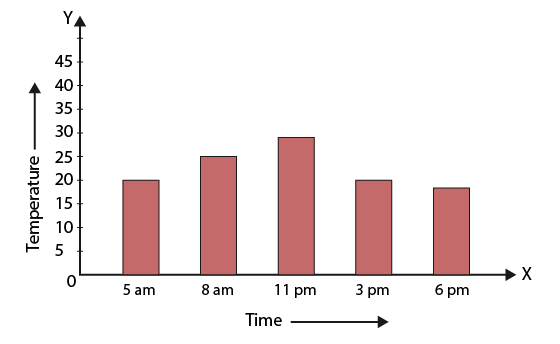3. The approximate velocities of some vehicles are given below:

 Name of vehicle Velocity (in km/ hr) Bicycle 27 Scooter 45 Car 90 Bus 72 Train 63

Draw a bar graph to represent the above data.

Solution:

Take vehicles on the X axis and the velocity on Y axis.

Consider 1 small square = 10 units on the Y axis

The bars should be of same width and space should be sufficient between consecutive bars.

The bar graph is as given below: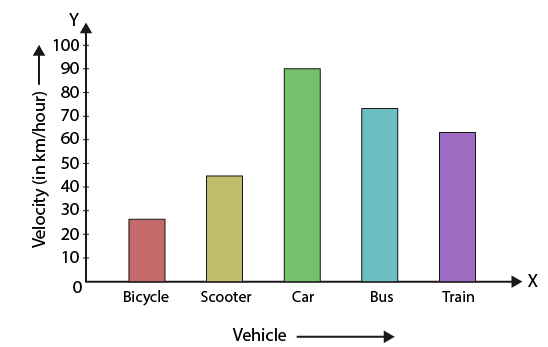4. The following table shows the favourite sports of 250 students of a school. Represent the data by a bar graph.

 Sports Number of students Cricket 75 Football 35 Tennis 50 Badminton 25 Swimming 65

Solution:

Take types of sports on the X axis and the number of students on Y axis.

Consider 1 small square = 10 units on the Y axis

The bars should be of same width and space should be sufficient between consecutive bars.

The bar graph is as given below: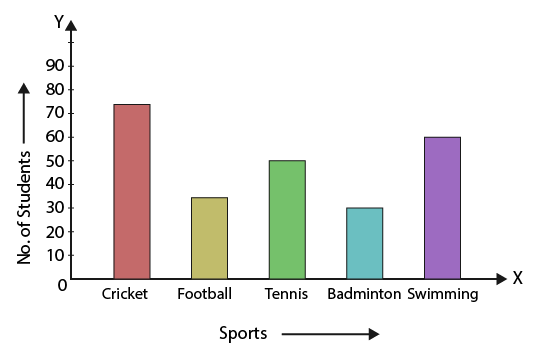5. Given below is a table which shows the year wise strength of a school. Represent this data by a bar graph.

 Year Number of students 2012-13 800 2013-14 975 2014-15 1100 2015-16 1400 2016-17 1625

Solution:

Take year on the X axis and the number of students on Y axis.

Consider 1 big division = 200 units on the Y axis

The bars should be of same width and space should be sufficient between consecutive bars.

The bar graph is as given below: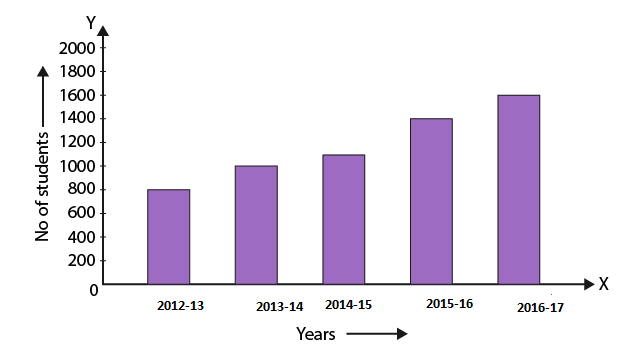6. The following table shows the number of scooters sold by a dealer during six consecutive years. Draw a bar graph to represent this data.

 Year Number of scooters sold (in thousands) 2011 16 2012 20 2013 32 2014 36 2015 40 2016 48

Solution:

Take year on the X axis and the number of scooters sold on Y axis.

Consider 1 small square = 10 units on the Y axis

The bars should be of same width and space should be sufficient between consecutive bars.

The bar graph is as given below: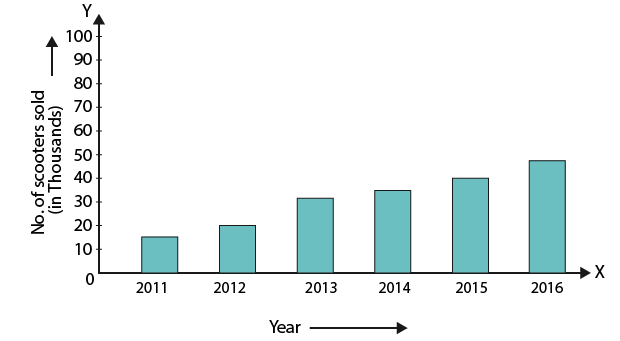7. The air distances of four cities from Delhi (in km) are given below:

 City Distance from Delhi (in km) Kolkata 1340 Mumbai 1100 Chennai 1700 Hyderabad 1220

Draw a bar graph to represent the above data.

Solution:

Take city on the X axis and the distance from Delhi on Y axis.

Consider 1 big division = 200 units on the Y axis

The bars should be of same width and space should be sufficient between consecutive bars.

The bar graph is as given below: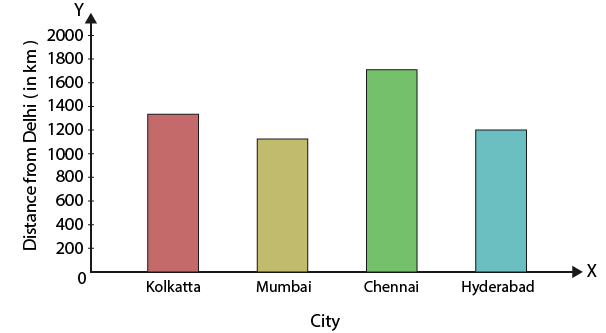8. The birth rate per thousand in five countries over a period of time is shown below:

 Country Birth rate per thousand China 42 India 35 Germany 14 UK 28 Sweden 21

Represent the above data by a bar graph.

Solution:

Take countries on the X axis and the birth rate on Y axis.

Consider 1 big division = 5 units on the Y axis

The bars should be of same width and space should be sufficient between consecutive bars.

The bar graph is as given below: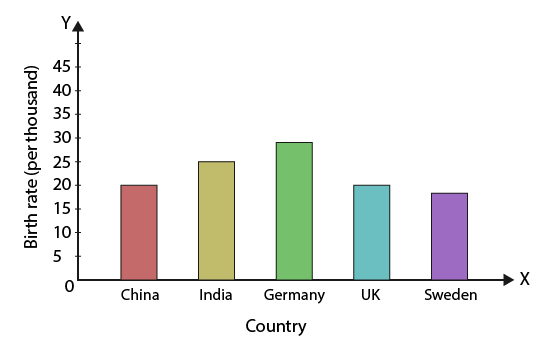9. The following table shows the life expectancy (average age to which people live in various countries in a particular year. Represent the data by a bar graph.

 Country Life expectancy (in years) Japan 84 India 68 Britain 80 Ethiopia 64 Cambodia 62 UK 73

Solution:

Take country on the X axis and the life expectancy on Y axis.

Consider 1 small square = 10 units on the Y axis

The bars should be of same width and space should be sufficient between consecutive bars.

The bar graph is as given below: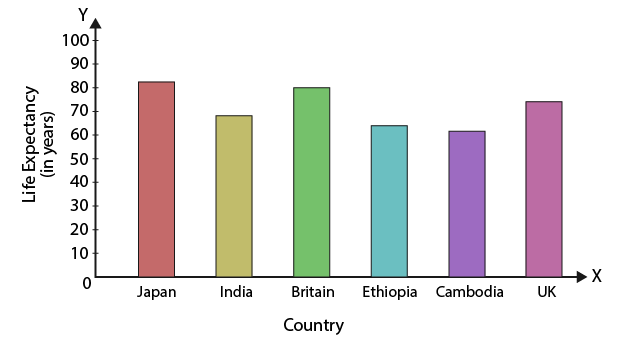10. Given below are the seats won by different political parties in the polling outcome of a state assembly elections:

 Political Party Seats won A 65 B 52 C 34 D 28 E 10 F 31

Draw a bar graph to represent the polling results.

Solution:

Take political party on the X axis and the seats won on Y axis.

Consider 1 small square = 10 units on the Y axis

The bars should be of same width and space should be sufficient between consecutive bars.

The bar graph is as given below: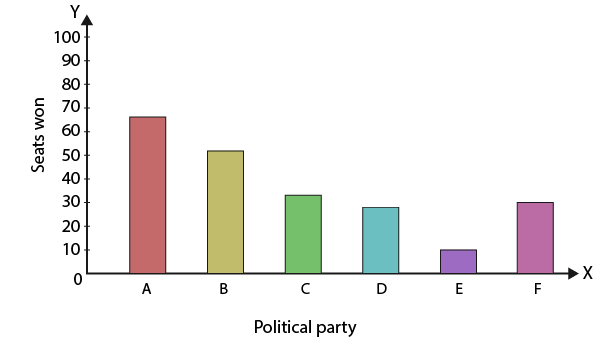11. Various modes of transport used by 185 students of a school are given below:

 School bus Private bus Bicycle Rickshaw By foot 640 360 490 210 150

Draw a bar graph to represent the above data.

Solution:

Take mode of transport on the X axis and the number of students on Y axis.

Consider 1 big division = 100 units on the Y axis

The bars should be of same width and space should be sufficient between consecutive bars.

The bar graph is as given below: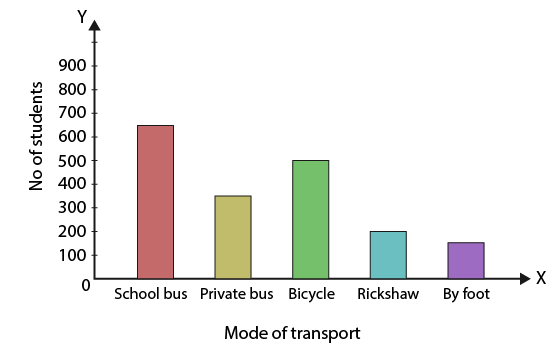12. Look at the bar graph given below: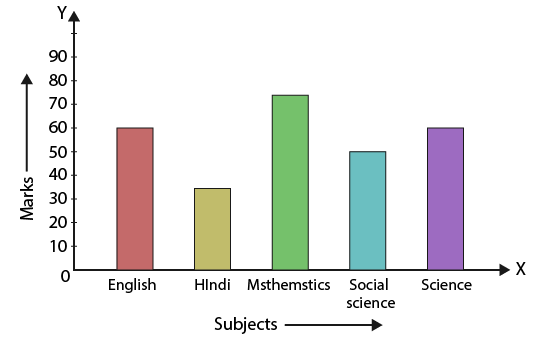(i) What information does the bar graph give?

(ii) In which subject is the student very good?

(iii) In which subject is he poor?

(iv) What is the average of his marks?

Solution:

(i) The bar graph shows the marks obtained by a student in various subjects in an examination.

(ii) The student is very good in mathematics.

(iii) He is poor in Hindi.

(iv) Average marks = (60 + 35 + 75 + 50 + 60)/ 5 = 280/5 = 56.

## Exercise 17(B) page: 658

1. The daily wages of 50 workers in a factory are given below:

 Daily wages (in ₹) Number of workers 340 – 380 16 380 – 420 9 420 – 460 12 460 – 500 2 500 – 540 7 540 – 580 4

Construct a histogram to represent the above frequency distribution.

Solution:

The frequency distribution is as follows:

 Daily wages (in ₹) Number of workers 340 – 380 16 380 – 420 9 420 – 460 12 460 – 500 2 500 – 540 7 540 – 580 4

We know that of the upper limit of one class is the lower limit of next class then exclusive method of classification is necessary. So the frequency distribution is in the exclusive form.

Consider the class intervals that is Daily wages (in ₹) on the X – axis and number of workers on the Y- axis and draw rectangles which provides the required histogram.

A break is indicated near the origin on the X axis since the scale starts at 340.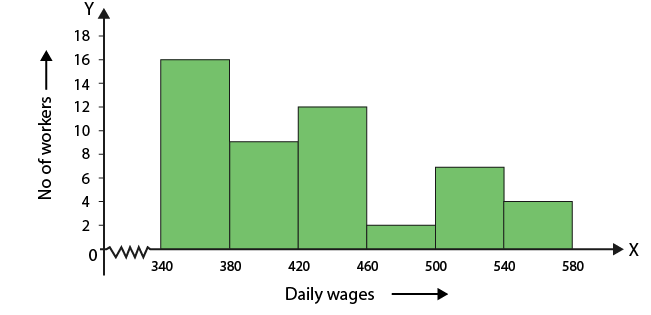2. The following table shows the average daily earnings of 40 general stores in a market, during a certain week:

 Daily earning (in rupees) Number of stores 700 – 750 6 750 – 800 9 800 – 850 2 850 – 900 7 900 – 950 11 950 – 1000 5

Draw a histogram to represent the above data.

Solution:

The frequency distribution is as follows:

 Daily earning (in rupees) Number of stores 700 – 750 6 750 – 800 9 800 – 850 2 850 – 900 7 900 – 950 11 950 – 1000 5

We know that of the upper limit of one class is the lower limit of next class then exclusive method of classification is necessary. So the frequency distribution is in the exclusive form.

Consider the class intervals that is Daily earning in rupees on the X – axis and number of stores on the Y- axis and draw rectangles which provides the required histogram.

A break is indicated near the origin on the X axis since the scale starts at 700.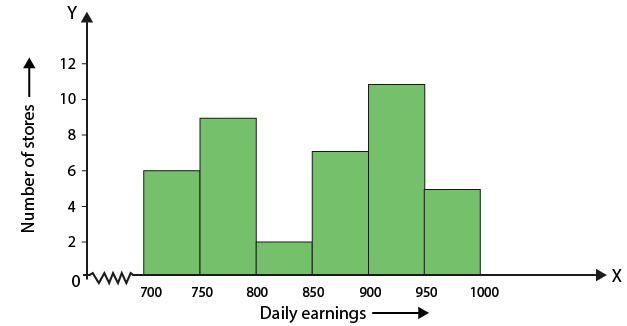3. The heights of 75 students in a school are given below:

 Height (in cm) Number of students 130 – 136 9 136 – 142 12 142 – 148 18 148 – 154 23 154 – 160 10 160 – 166 3

Draw a histogram to represent the above data.

Solution:

The frequency distribution is as follows:

 Height (in cm) Number of students 130 – 136 9 136 – 142 12 142 – 148 18 148 – 154 23 154 – 160 10 160 – 166 3

We know that of the upper limit of one class is the lower limit of next class then exclusive method of classification is necessary. So the frequency distribution is in the exclusive form.

Consider the class intervals that is height in cm on the X – axis and number of students on the Y- axis and draw rectangles which provides the required histogram.

A break is indicated near the origin on the X axis since the scale starts at 130.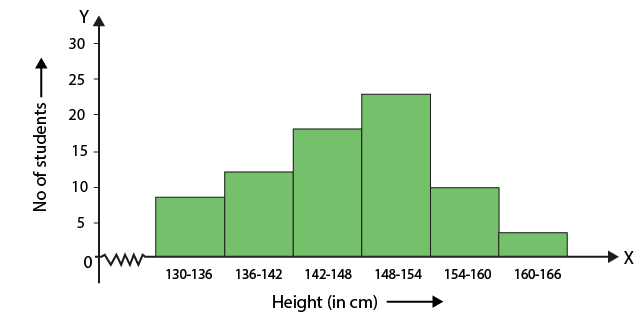4. The following table gives the lifetimes of 400 neon lamps:

 Lifetime (in hr) Number of lamps 300 – 400 14 400 – 500 56 500 – 600 60 600 – 700 86 700 – 800 74 800 – 900 62 900 – 1000 48

(i) Represent the given information with the help of a histogram.

(ii) How many lamps have a lifetime of more than 700 hours?

Solution:

(i) Histogram is given below: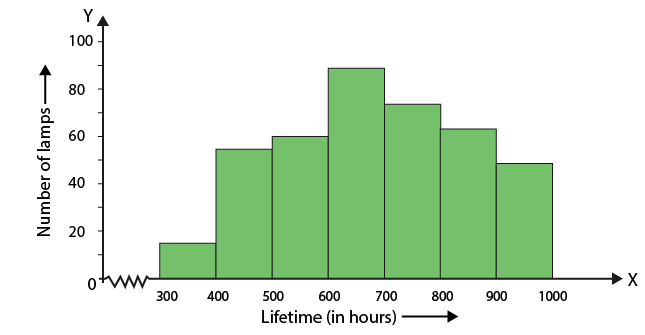(ii) Number of lamps having a lifetime of more than 700 hours = (74 + 62 + 48) = 184.

5. Draw a histogram for the frequency distribution of the following data:

 Class interval Frequency 8 – 13 320 13 – 18 780 18 – 23 160 23 – 28 540 28 – 33 260 33 – 38 100 38 – 43 80

Solution:

The frequency distribution is as follows:

 Class interval Frequency 8 – 13 320 13 – 18 780 18 – 23 160 23 – 28 540 28 – 33 260 33 – 38 100 38 – 43 80

We know that of the upper limit of one class is the lower limit of next class then exclusive method of classification is necessary. So the frequency distribution is in the exclusive form.

Consider the class intervals on the X – axis and frequency on the Y- axis and draw rectangles which provides the required histogram.

A break is indicated near the origin on the X axis since the scale starts at 8.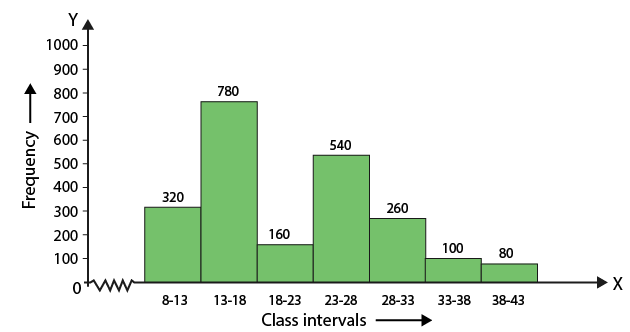6. Construct a histogram for the following frequency distribution:

 Class interval Frequency 5 – 12 6 13 – 20 15 21 – 28 24 29 – 36 18 37 – 44 4 45 – 52 9

Solution:

Convert the given inclusive form into an exclusive form by taking intervals as given below:

 Class interval Frequency 4.5 – 12.5 6 12.5 – 20.5 15 20.5 – 28.5 24 28.5 – 36.5 18 36.5 – 44.5 4 454.5 – 52.5 9

Consider the class intervals on the X – axis and frequency on the Y- axis and draw rectangles which provides the required histogram.

A break is indicated near the origin on the X axis since the scale starts at 4.5.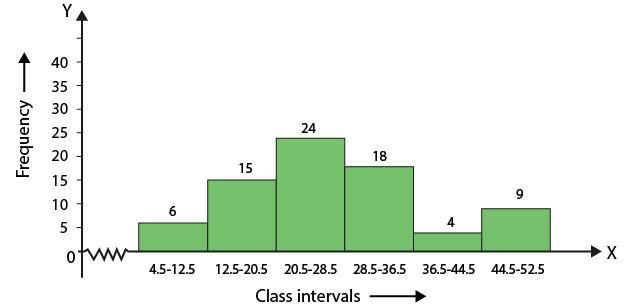7. The following table shows the number of illiterate persons in the age group (10 – 58 years) in a town:

 Age group (in years) Number of illiterate persons 10 – 16 175 17 – 23 325 24 – 30 100 31 – 27 150 38 – 44 250 45 – 51 400 52 – 58 525

Draw a histogram to represent the above data.

Solution:

Convert the given inclusive form into an exclusive form by taking intervals as given below:

 Age group (in years) Number of illiterate persons 9.5 – 16.5 175 16.5 – 23.5 325 23.5 – 30.5 100 30.5 – 37.5 150 37.5 – 44.5 250 44.5 – 51.5 400 51.5 – 58.5 525

Consider the class intervals that is age group in years on the X – axis and number of illiterate persons on the Y- axis and draw rectangles which provides the required histogram.

A break is indicated near the origin on the X axis since the scale starts at 9.5.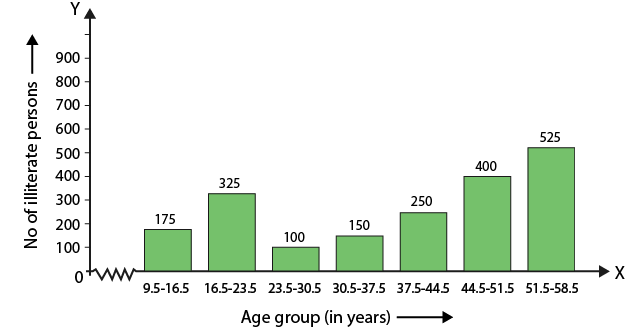8. Draw a histogram to represent the following data:

 Class interval Frequency 10 – 14 5 14 – 20 6 20 – 32 9 32 – 52 25 52 – 80 21

Solution:

The frequency distribution is as follows:

 Class interval Frequency 10 – 14 5 14 – 20 6 20 – 32 9 32 – 52 25 52 – 80 21

The class sizes are 4, 6, 12, 20 and 28

So the minimum class size is 4

We know that

Adjusted frequency = (minimum class size × its frequency)/ class size of this class

On further calculation we get

4/4 × 5 = 5

4/6 × 6 = 4

4/12 × 9 = 3

4/20 × 25 = 5

4/28 × 21 = 3

So the adjusted frequency table is given below:

 Class interval Frequency 10 – 14 5 14 – 20 4 20 – 32 3 32 – 52 5 52 – 80 3

Consider the class intervals on the X – axis and frequency on the Y- axis and draw rectangles which provides the required histogram.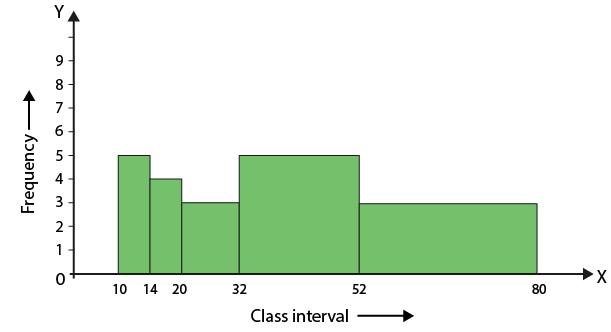9. 100 surnames were randomly picked up from a local telephone directory and frequency distribution of the number of letters in the English alphabet in the surnames was found as follows:

 Number of letters Number of surnames 1 – 4 6 4 – 6 30 6 – 8 44 8 – 12 16 12 – 20 4

(i) Draw a histogram to depict the given information.

(ii) Write the class interval in which the maximum number of surnames lie.

Solution:

(i) The frequency distribution is as follows:

 Number of letters Number of surnames 1 – 4 6 4 – 6 30 6 – 8 44 8 – 12 16 12 – 20 4

The class sizes are 3, 2, 2, 4 and 8

So the minimum class size is 2

We know that

Adjusted frequency = (minimum class size × its frequency)/ class size of this class

On further calculation we get

2/3 × 6 = 4

2/2 × 30 = 30

2/2 × 44 = 44

2/4 × 16 = 8

2/8 × 4 = 1

So the adjusted frequency table is given below:

 Number of letters Number of surnames 1 – 4 4 4 – 6 30 6 – 8 44 8 – 12 8 12 – 20 1

Consider the class intervals that is number of letters on the X – axis and number of surnames on the Y- axis and draw rectangles which provides the required histogram.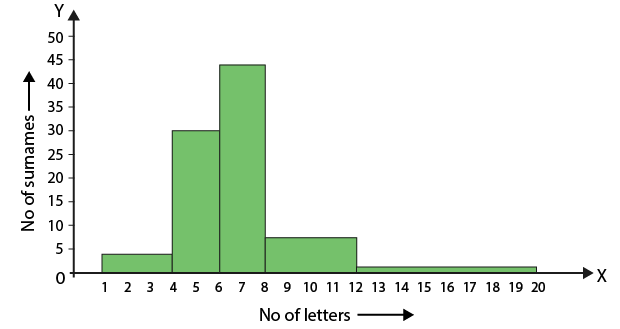(ii) Maximum surnames lie in class interval 6 – 8.

10. Draw a histogram to represent the following information:

 Class interval Frequency 5 – 10 6 10 – 15 12 15 – 25 10 25 – 45 8 45 – 75 18

Solution:

The frequency distribution is as follows:

 Class interval Frequency 5 – 10 6 10 – 15 12 15 – 25 10 25 – 45 8 45 – 75 18

The class sizes are 5, 5, 10, 2 and 30

So the minimum class size is 5

We know that

Adjusted frequency = (minimum class size × its frequency)/ class size of this class

On further calculation we get

5/5 × 6 = 6

5/5 × 12 = 12

5/10 × 10 = 5

5/20 × 8 = 2

5/30 × 18 = 3

So the adjusted frequency table is given below:

 Class interval Frequency 5 – 10 6 10 – 15 12 15 – 25 5 25 – 45 2 45 – 75 3

Consider the class intervals on the X – axis and frequency on the Y- axis and draw rectangles which provides the required histogram.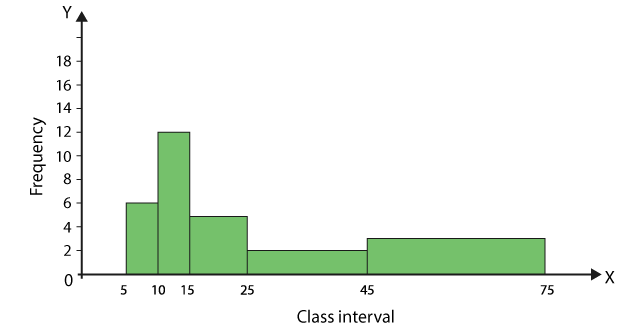11. Draw a histogram to represent the following information:

 Marks Number of students 0 – 10 8 10 – 30 32 30 – 45 18 45 – 50 10 50 – 60 6

Solution:

The frequency distribution is as follows:

 Marks Number of students 0 – 10 8 10 – 30 32 30 – 45 18 45 – 50 10 50 – 60 6

The class sizes are 10, 20, 15, 5 and 10.

So the minimum class size is 5

We know that

Adjusted frequency = (minimum class size × its frequency)/ class size of this class

On further calculation we get

5/10 × 8 = 4

5/20 × 32 = 8

5/15 × 18 = 6

5/5 × 10 = 10

5/10 × 6 = 3

So the adjusted frequency table is given below:

 Marks Number of students 0 – 10 4 10 – 30 8 30 – 45 6 45 – 50 10 50 – 60 3

Consider the class intervals that is marks on the X – axis and number of students on the Y- axis and draw rectangles which provides the required histogram.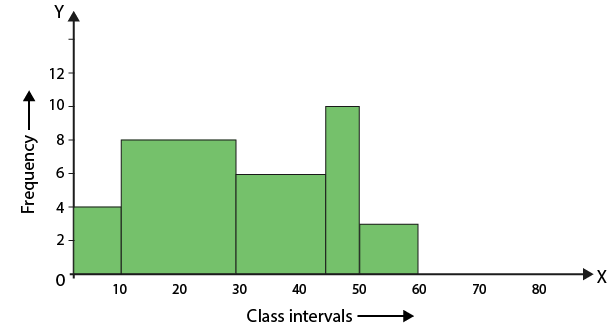12. In a study of diabetic patients in a village, the following observations were noted.

 Age in years Number of patients 10 – 20 2 20 – 30 5 30 – 40 12 40 – 50 19 50 – 60 9 60 – 70 4

Represent the above data by a frequency polygon.

Solution:

The frequency distribution is as follows:

 Age in years Number of patients 10 – 20 2 20 – 30 5 30 – 40 12 40 – 50 19 50 – 60 9 60 – 70 4

We know that

Class mark of a class interval = (lower limit + upper limit)/ 2

 Age in years Class marks Frequency 0 – 10 5 0 10 – 2 15 2 20 – 30 25 5 30 – 40 35 12 40 – 50 45 19 50 – 60 55 9 60 – 70 65 4 70 – 80 75 0

By taking imaginary class intervals 0 – 10 and 70 – 80 with frequency 0 plot the points by taking class marks on X-axis and frequencies on the Y-axis.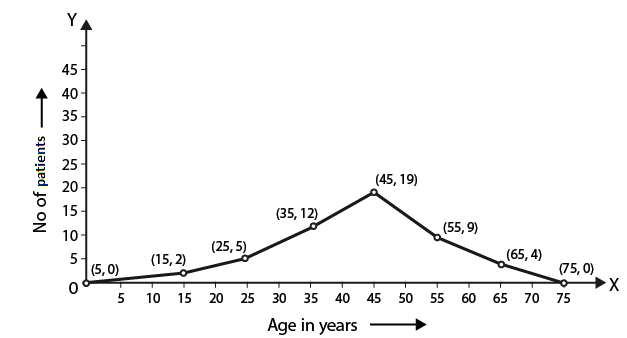13. Draw a frequency polygon for the following frequency distribution:

 Class interval Frequency 1 – 10 8 11 – 20 3 21 – 30 6 31 – 40 12 41 – 50 2 51 – 60 7

Solution:

The frequency distribution is as follows:

 Class interval Frequency 1 – 10 8 11 – 20 3 21 – 30 6 31 – 40 12 41 – 50 2 51 – 60 7

Convert the given inclusive form into an exclusive form by taking intervals as 0.5 – 1.5, 10.5 – 20.5, 20.5 – 30.5, 40.5 – 40.5, 40.5 – 50.5 and 50.5 – 60.5

We know that

Class mark of a class interval = (lower limit + upper limit)/ 2

Consider – 9.5 – 0.5 and 60.5 – 70.5 with frequency zero the table is as follows:

 Class interval True class intervals Class marks Frequency (-9) – 0 (-9.5) – 0.5 -4.5 0 1 – 10 0.5 – 10.5 5.5 8 11 – 20 10.5 – 20.5 15.5 3 21 – 30 20.5 – 30.5 25.5 6 31 – 40 30.5 – 40.5 35.5 12 41 – 50 40.5 – 50.5 45.4 2 51 – 60 50.5 – 60.5 55.5 7 61 – 70 60.5 – 70.5 65.5 0

Consider the class intervals that is marks on the X – axis and frequency on the Y- axis.

Plot the points and join them to obtain the frequency polygon.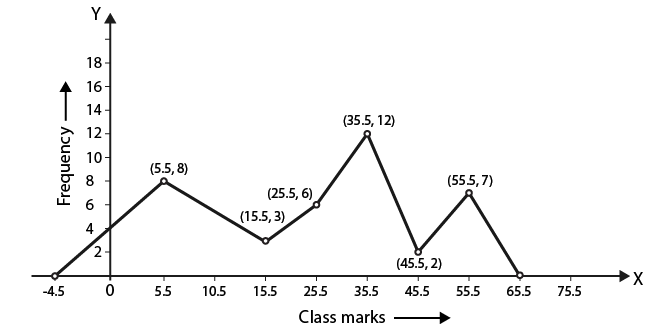14. The age (in years) of 360 patients treated in a hospital on a particular day are given below:

 Age in years Number of patients 10 – 20 90 20 – 30 40 30 – 40 60 40 – 50 20 50 – 60 120 60 – 70 30

Draw a histogram and frequency polygon on the same graph to represent the above data.

Solution:

The frequency distribution is as follows:

 Age in years Number of patients 10 – 20 90 20 – 30 40 30 – 40 60 40 – 50 20 50 – 60 120 60 – 70 30

Consider the class intervals that is age in years on the X – axis and number of patients on the Y- axis and draw rectangles which provides the required histogram.

To construct a frequency polygon, take 0 – 10 and 70 – 80 as imaginary class intervals having class marks 5 and 75 with frequency zero and join the midpoints of rectangles i.e. A (5, 0) and B (75, 0).

So the frequency polygon is as given below: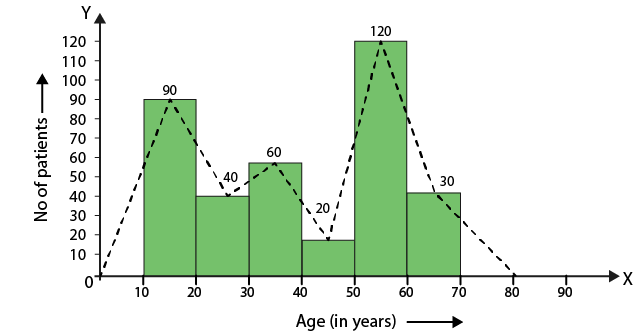15. Draw a histogram and the frequency polygon from the following data:

 Class interval Frequency 20 – 25 30 25 – 30 24 30 – 35 52 35 – 40 28 40 – 45 46 45 – 50 10

Solution:

The frequency distribution is as follows:

 Class interval Frequency 20 – 25 30 25 – 30 24 30 – 35 52 35 – 40 28 40 – 45 46 45 – 50 10

Consider the class intervals on the X – axis and frequency on the Y- axis and draw rectangles which provides the required histogram.

To construct a frequency polygon, take 15 – 20 and 50 – 55 as imaginary class intervals with frequency zero and join the midpoints of rectangles.

So the frequency polygon is as given below: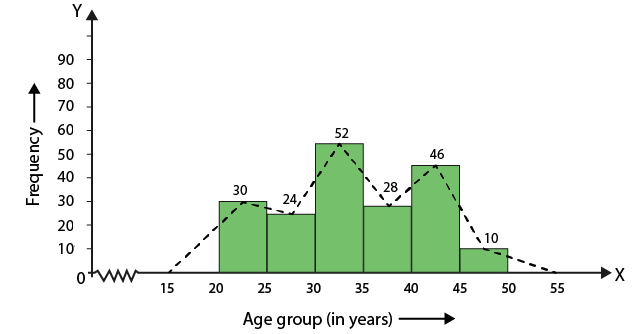16. Draw a histogram for the following data:

 Class interval Frequency 600 – 640 18 640 – 680 45 680 – 720 153 720 – 760 288 760 – 800 171 800 – 840 63

Using this histogram, draw the frequency polygon on the same graph.

Solution:

The frequency distribution is as follows:

 Class interval Frequency 600 – 640 18 640 – 680 45 680 – 720 153 720 – 760 288 760 – 800 171 800 – 840 63

Consider the class intervals on the X – axis and frequency on the Y- axis and draw rectangles which provides the required histogram.

To construct a frequency polygon, take 560 – 600 and 840 – 880 as imaginary class intervals with frequency zero and join the midpoints of rectangles.

So the frequency polygon is as given below: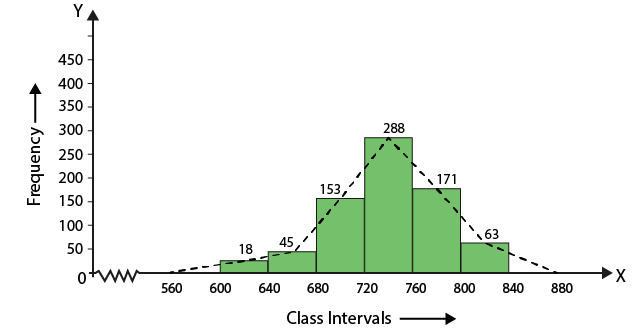### RS Aggarwal Solutions for Class 9 Maths Chapter 17: Bar Graph, Histogram and Frequency Polygon

Chapter 17, Bar Graph, Histogram and Frequency Polygon, has 2 exercises with problems based on graphical representation of the given data. The concepts which are discussed in RS Aggarwal Solutions Chapter 17 are given below:

• Bar Graph
• Bar Graph or Column Graph